# Concise Selina Solutions for Class 9 Maths Chapter 12

The Selina Solutions for the questions given in Chapter 12, Mid-Point and Its Converse, of the Class 9 Selina textbooks are available here. In this chapter students learn about the topic of Mid-Point and Its Converse of a triangle as well as the intercept theorem in detail. Students can easily score full marks in the exams by solving all the questions present in the Selina textbook.

The Class 9 Selina Solutions Maths is very easy to understand. These solutions cover all the exercise questions included in the book and are according to the syllabus prescribed by the ICSE or CISCE. Here, the PDF of the Class 9 Maths Chapter 12 Selina Solutions is available, which can be downloaded as well as viewed online. Students can also avail the Selina Solutions and download it for free to practice offline as well.

### Download PDF of Selina Solutions for Class 9 Maths Chapter 12:-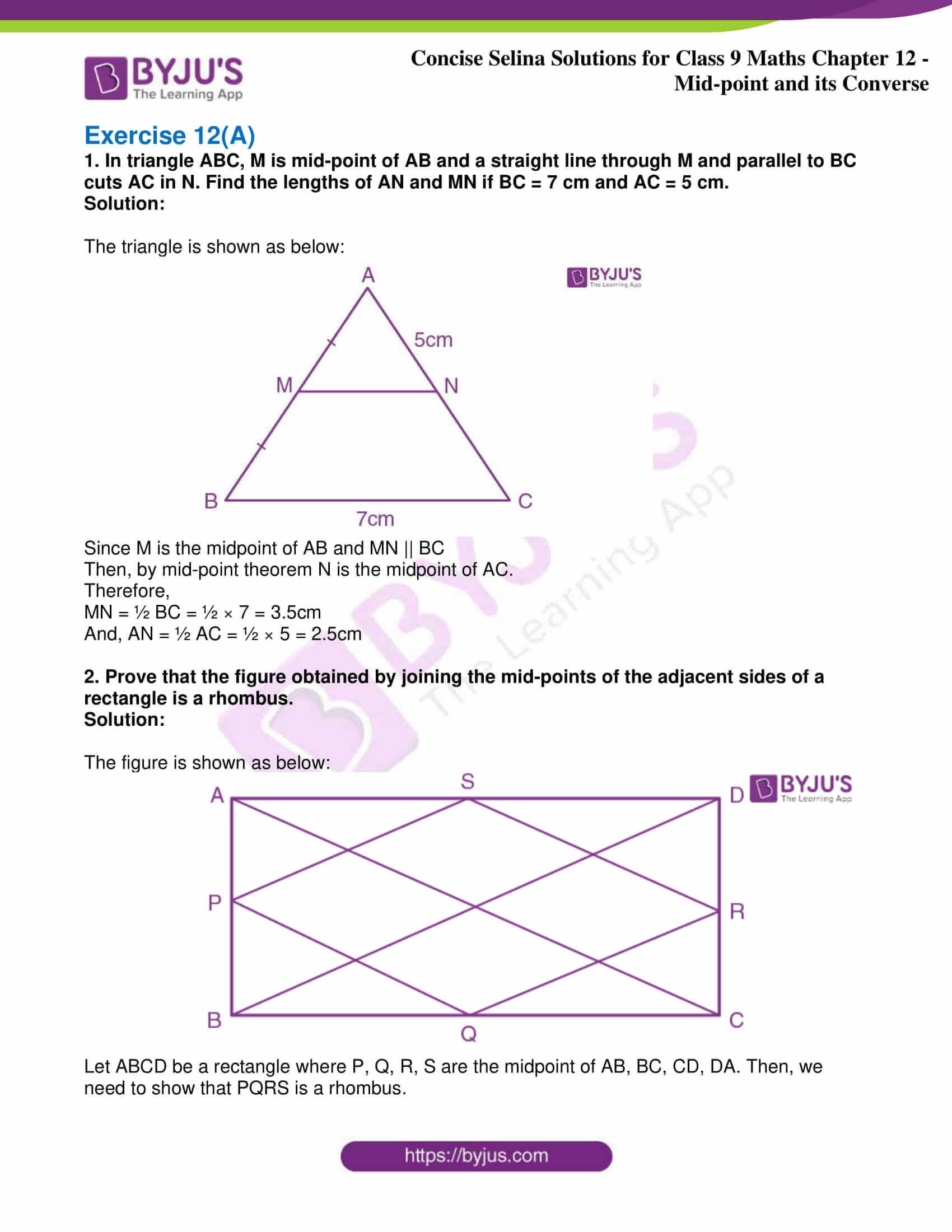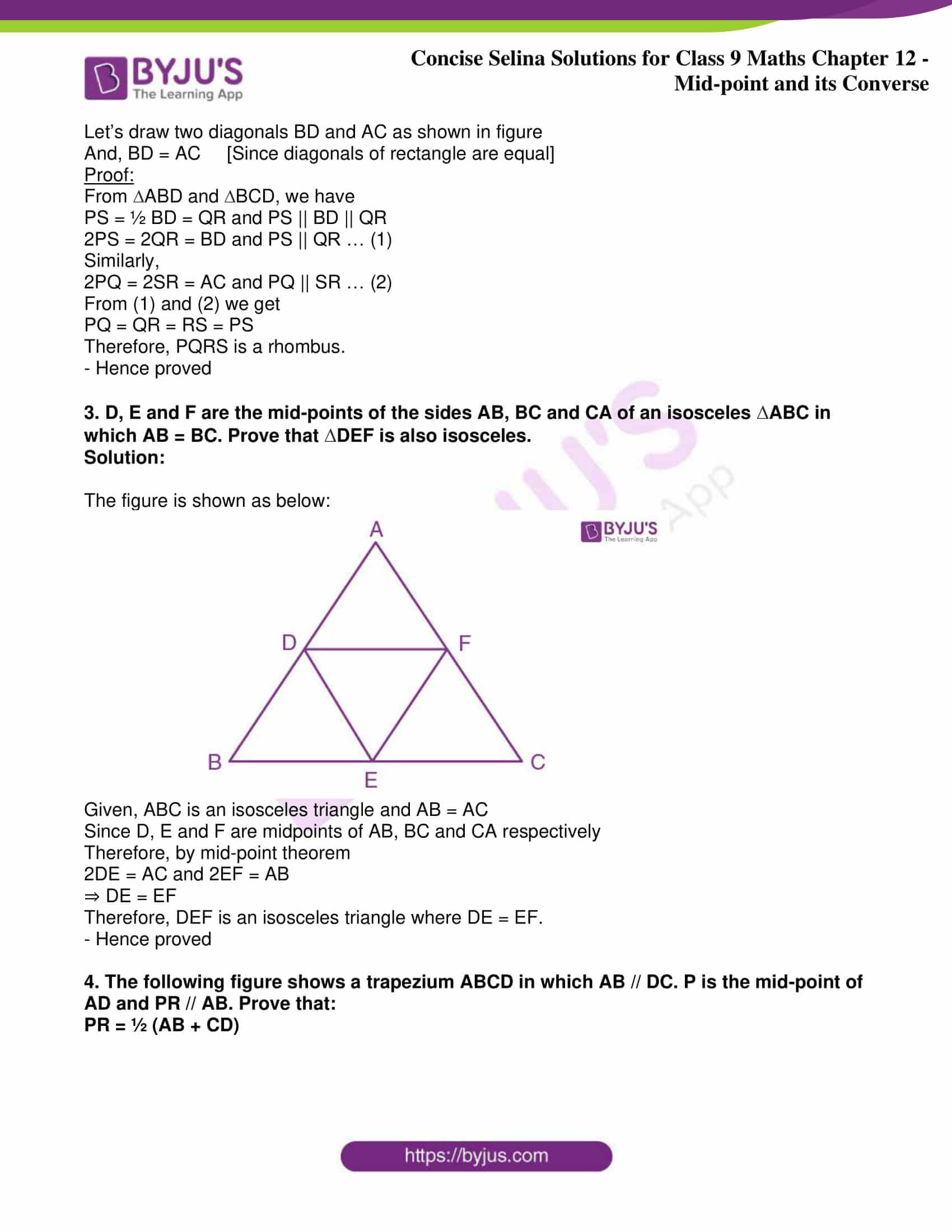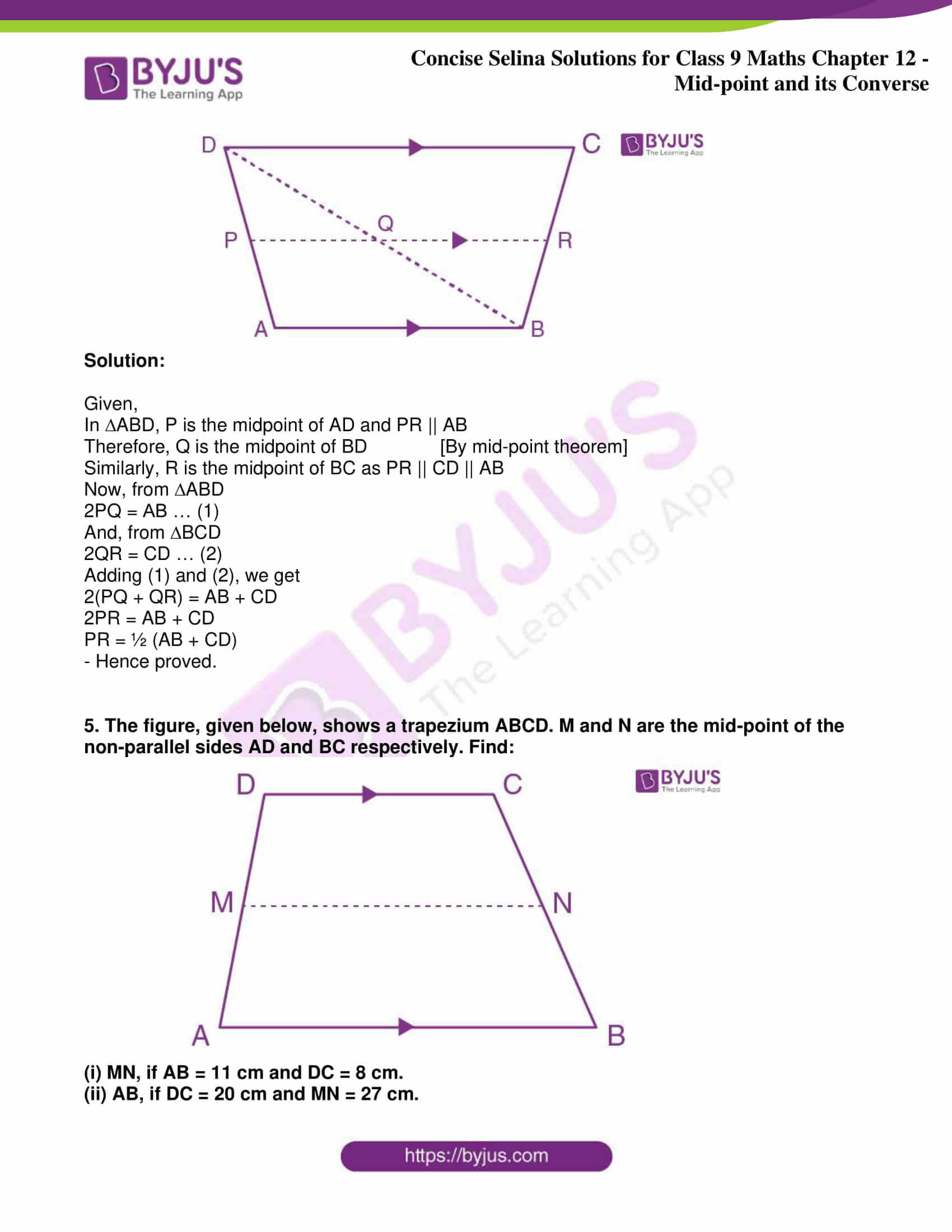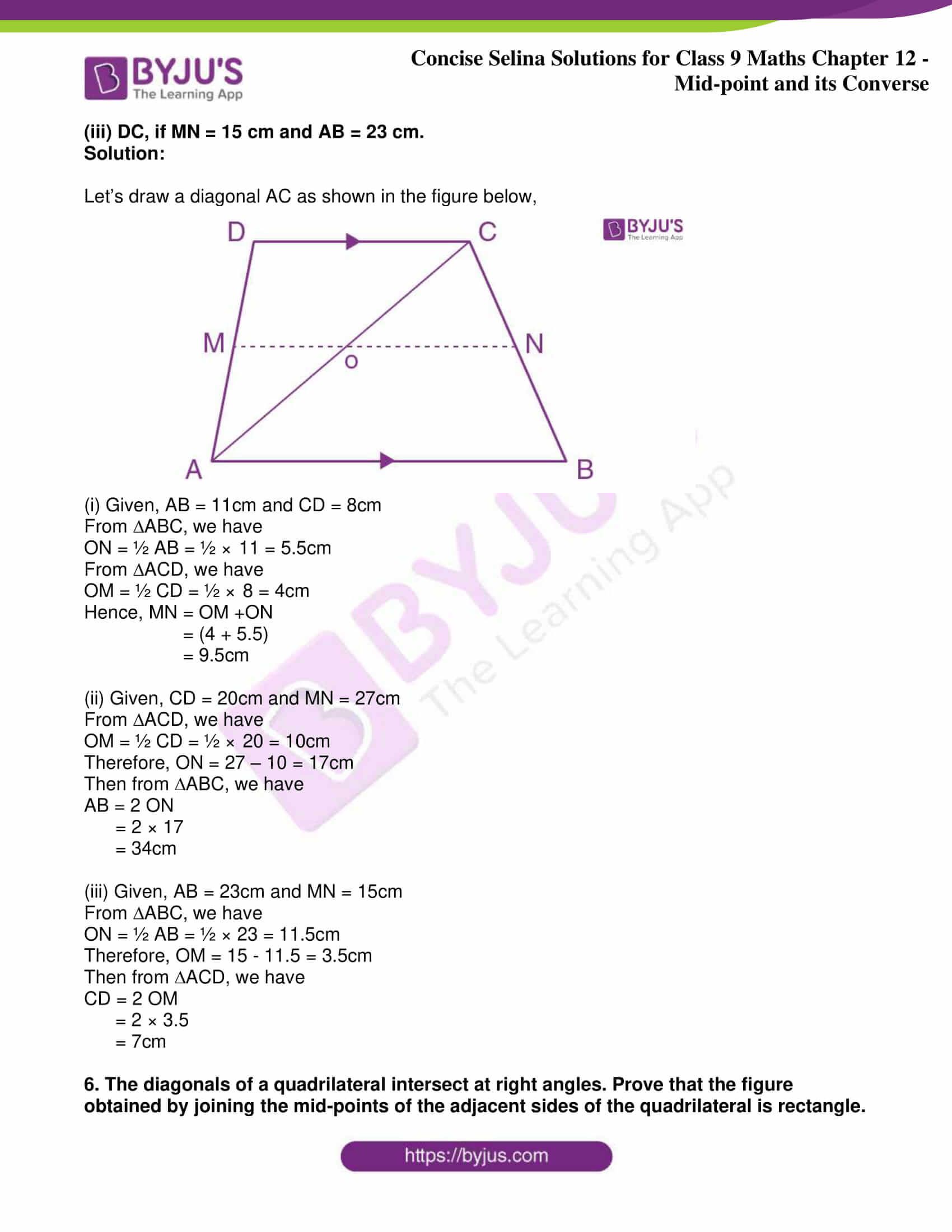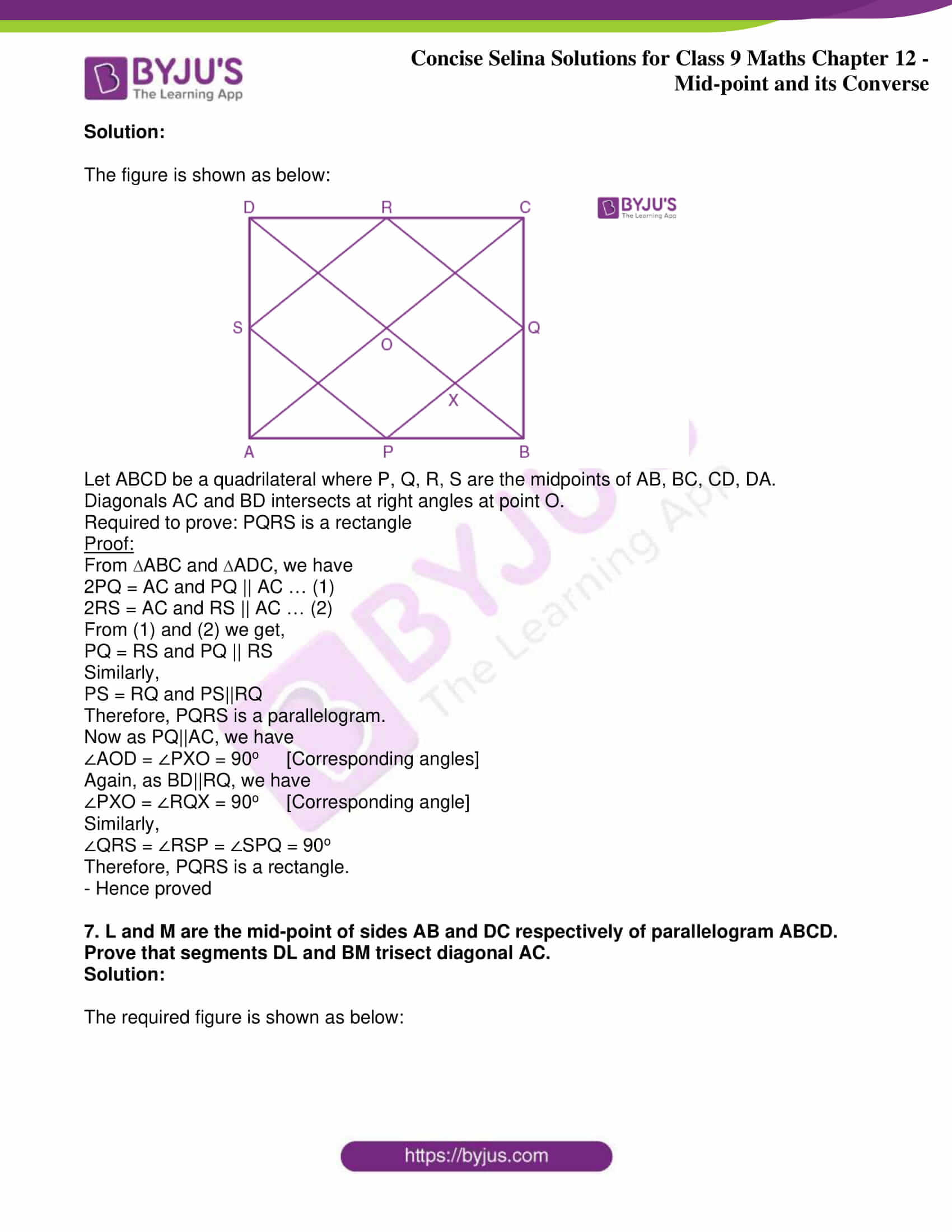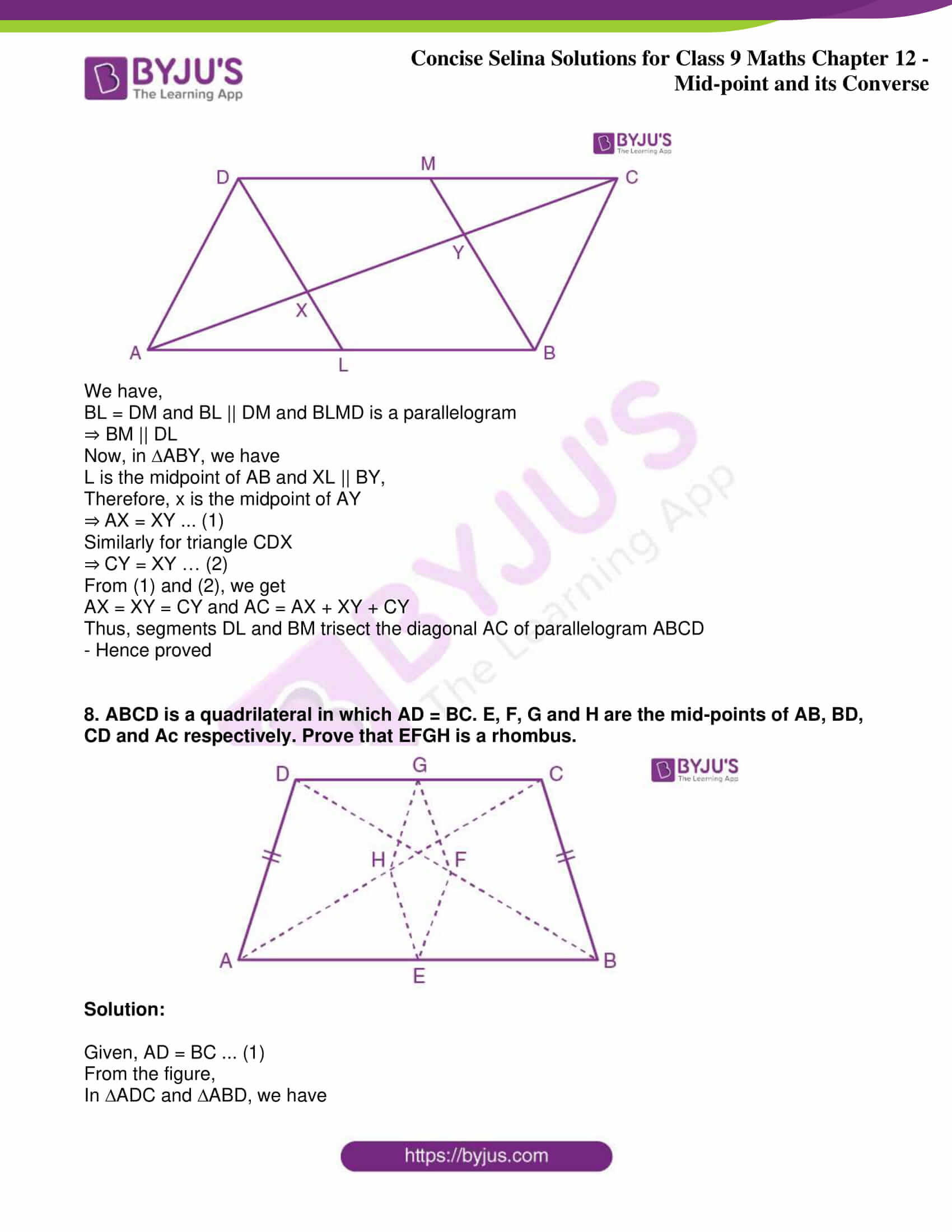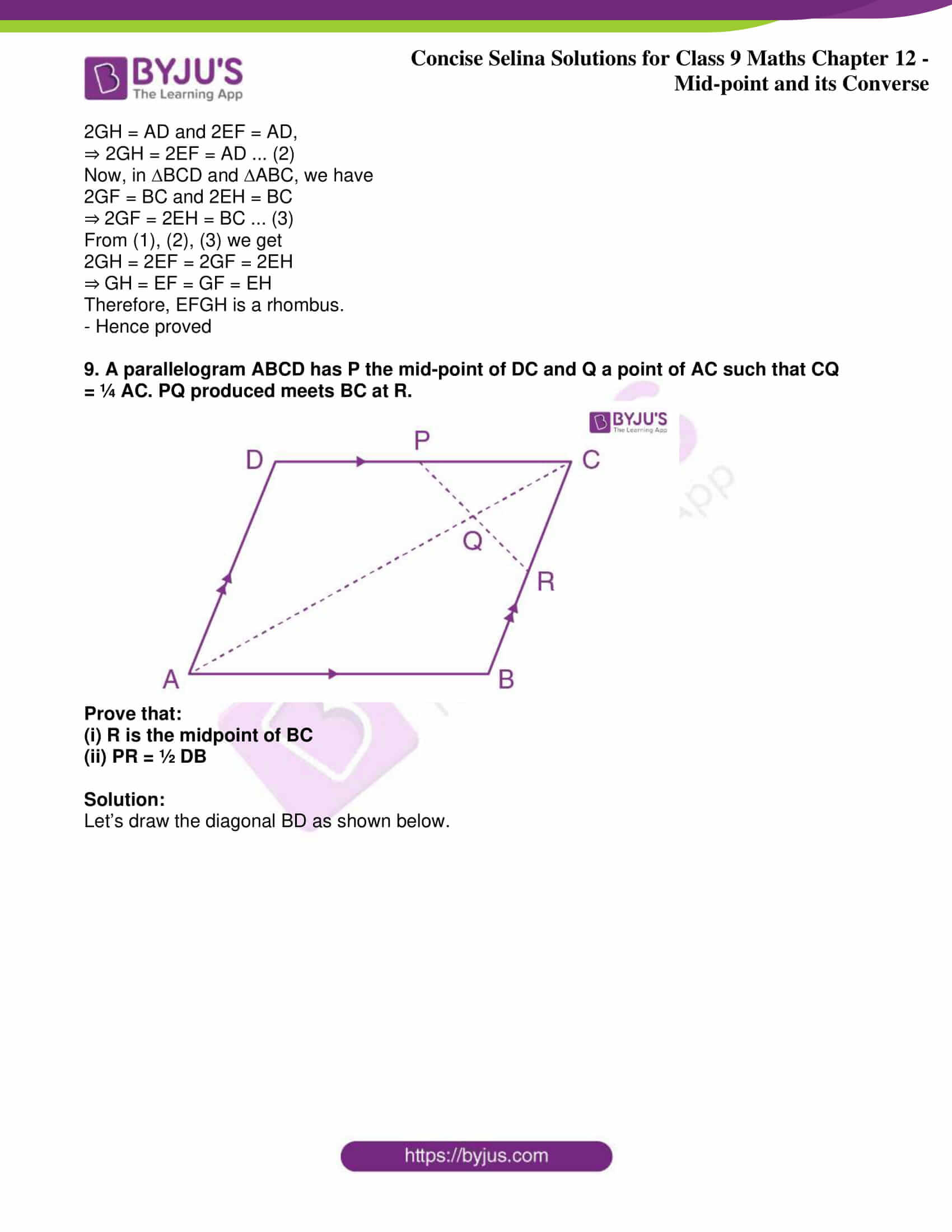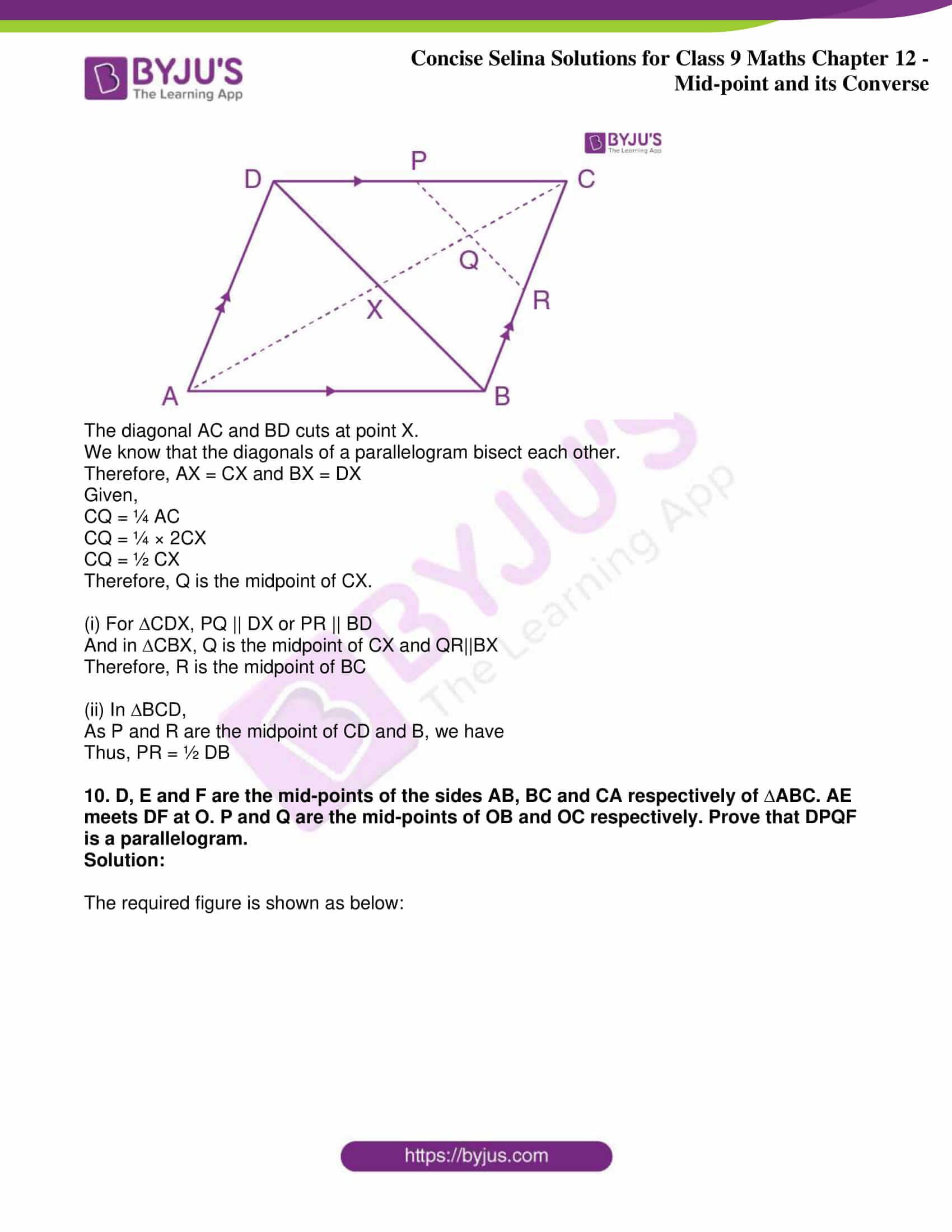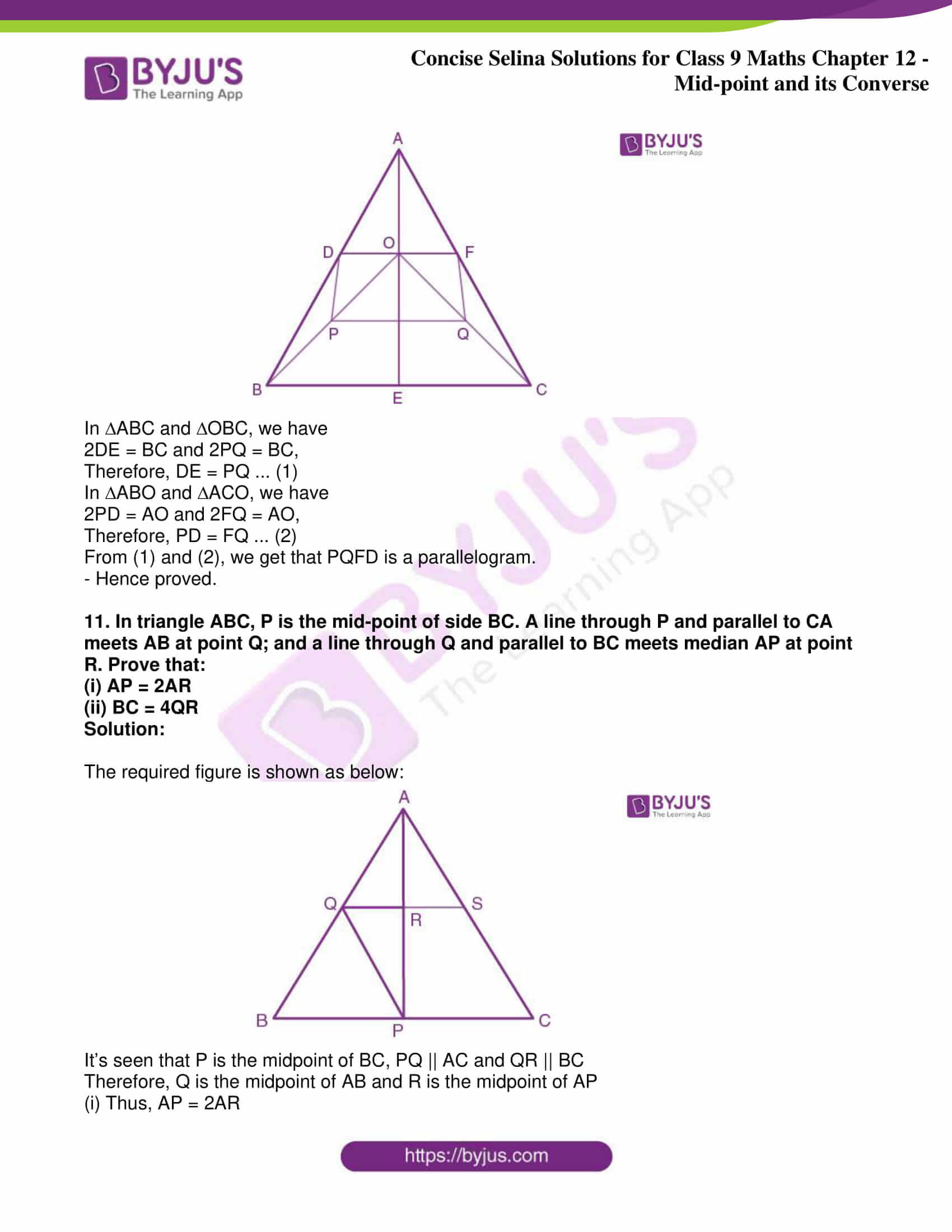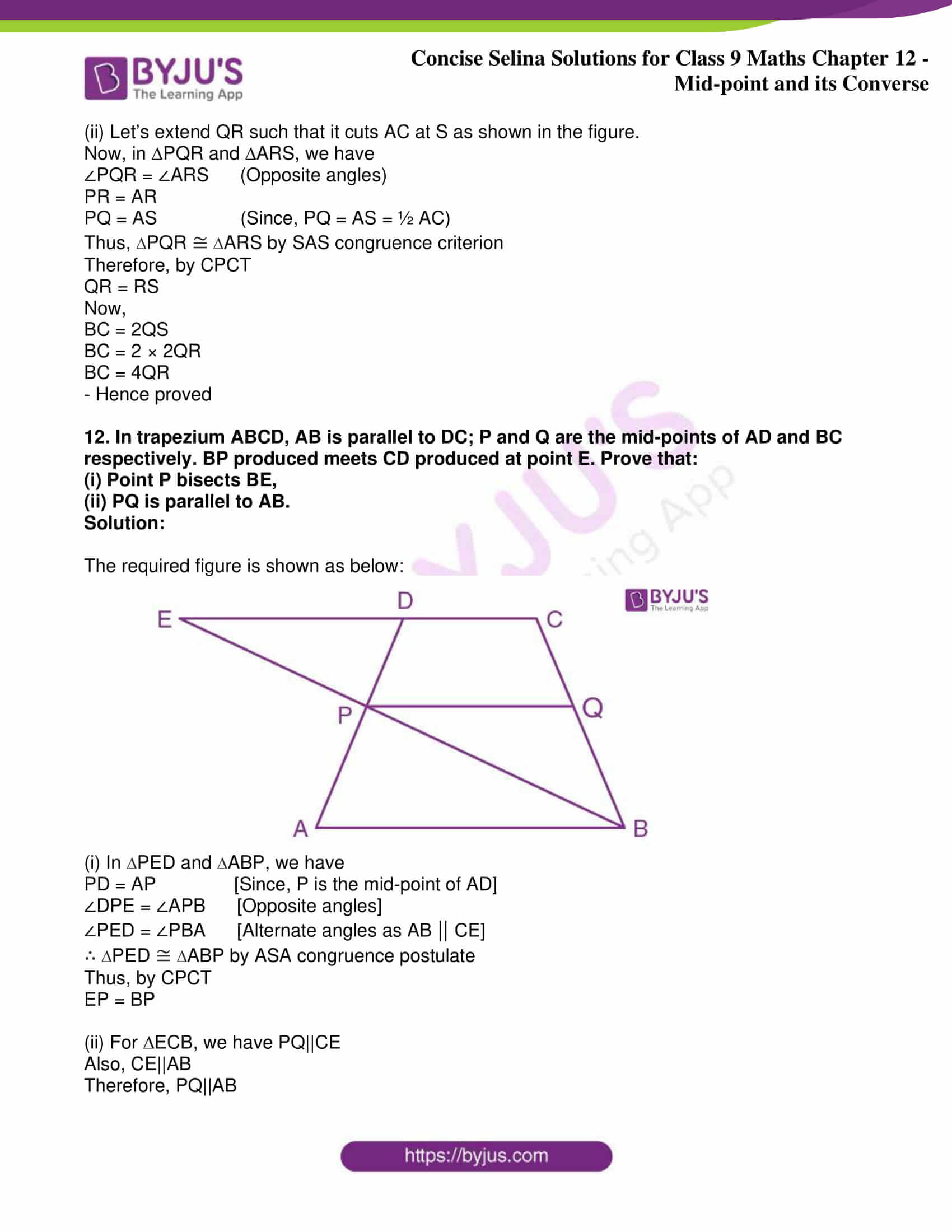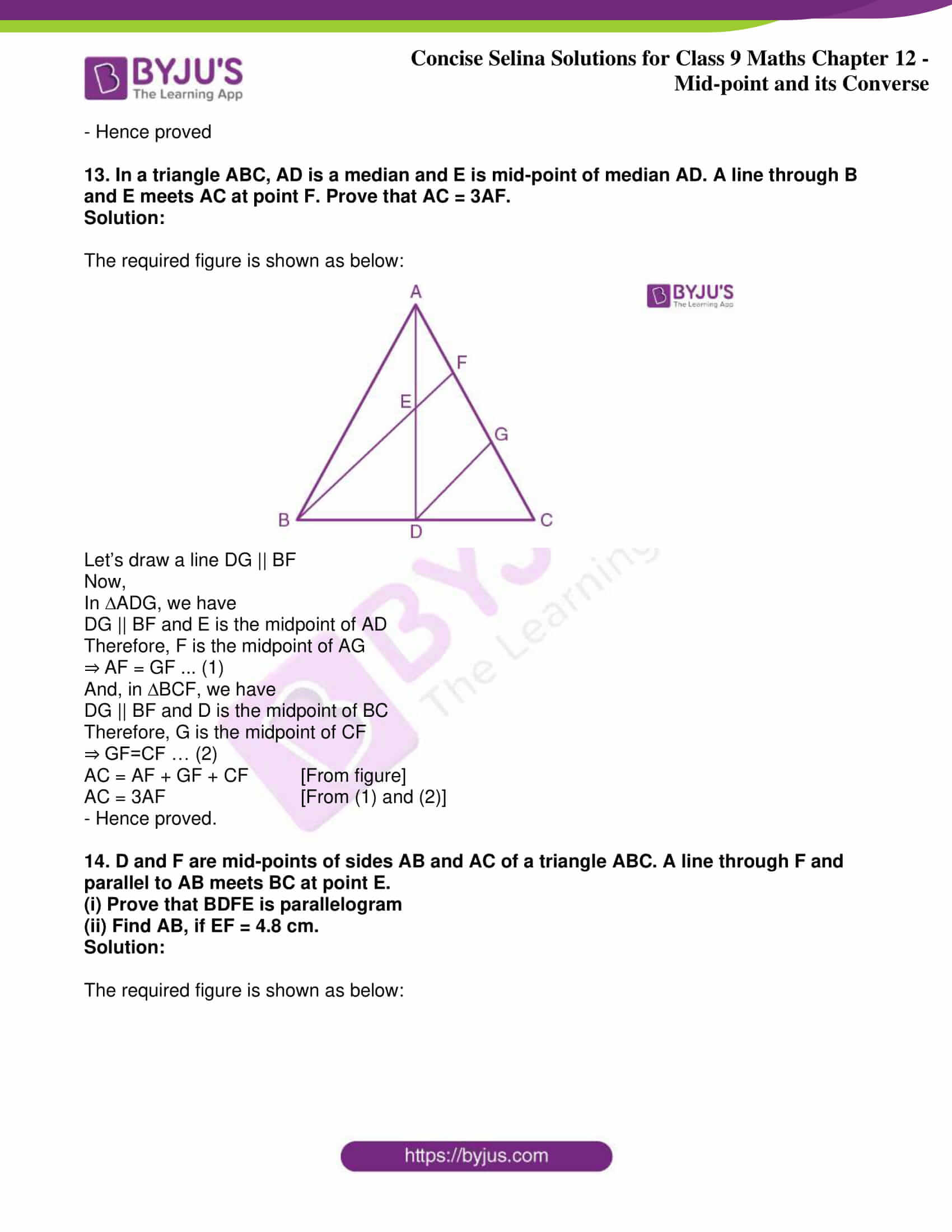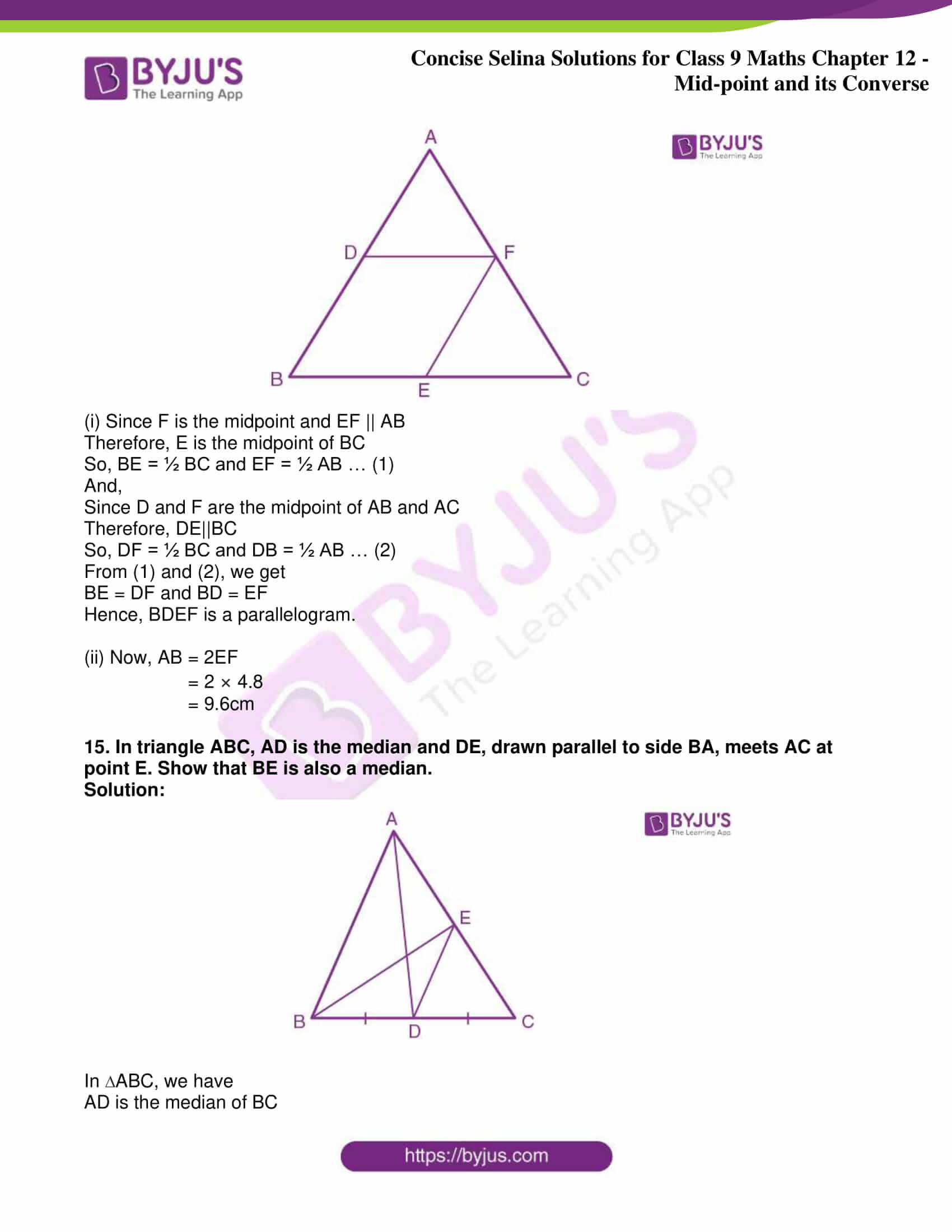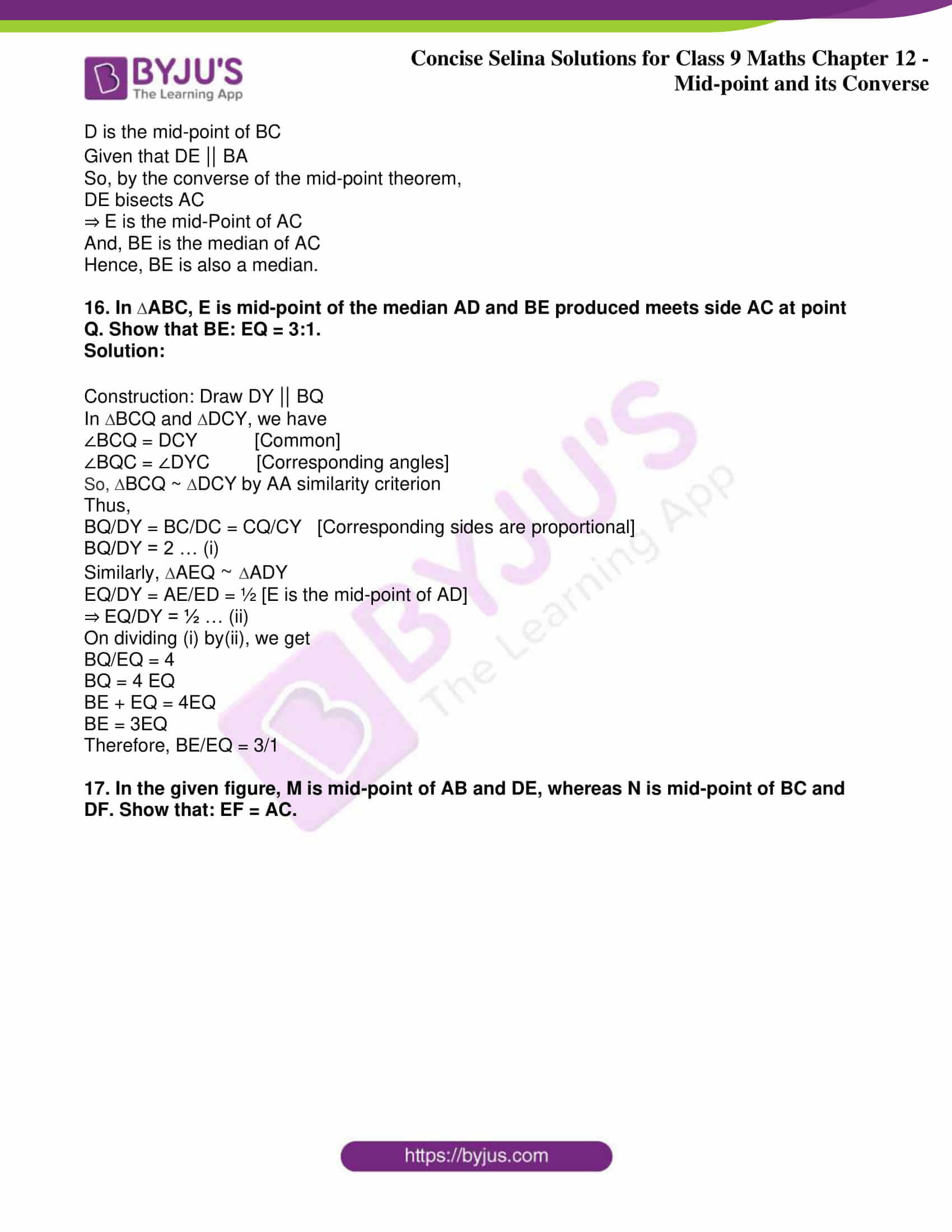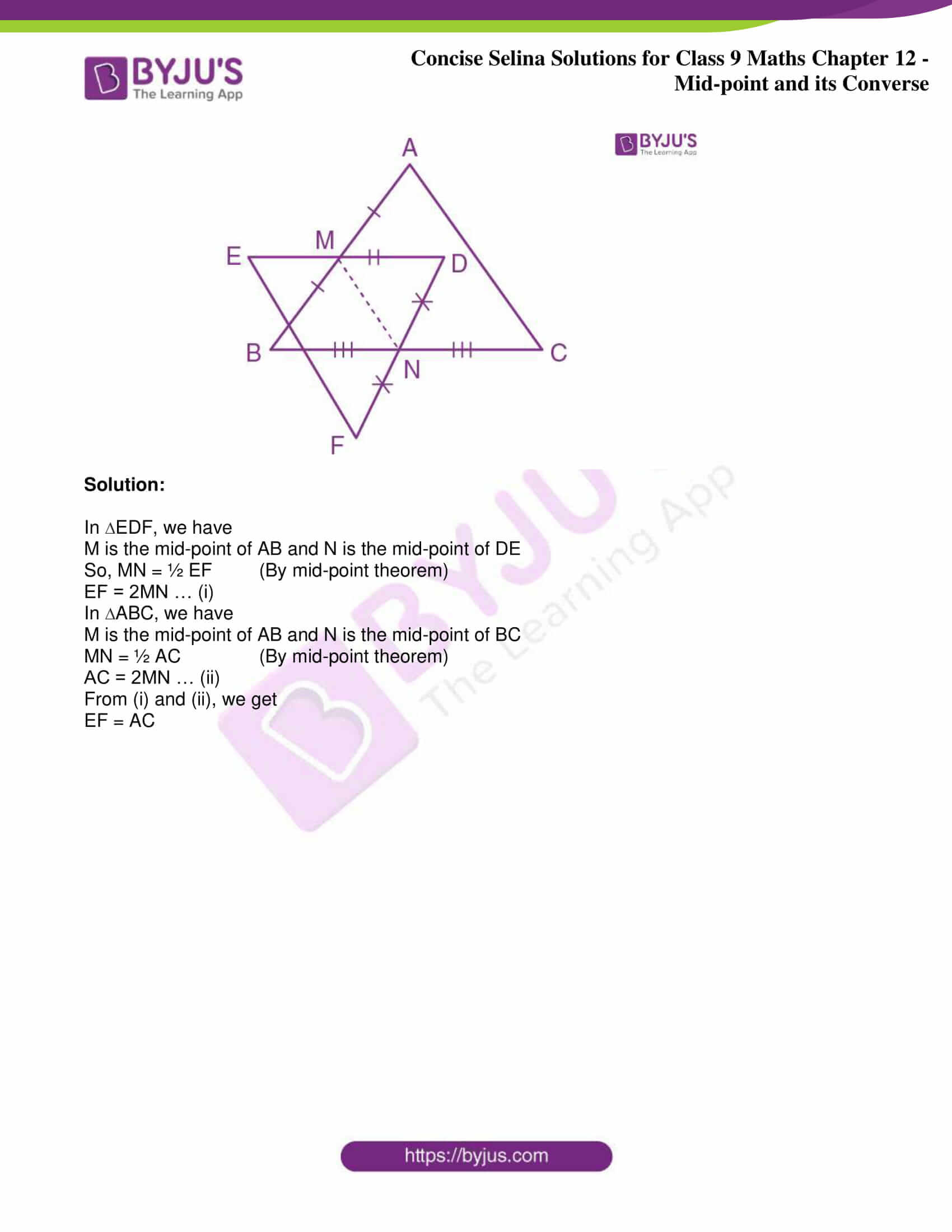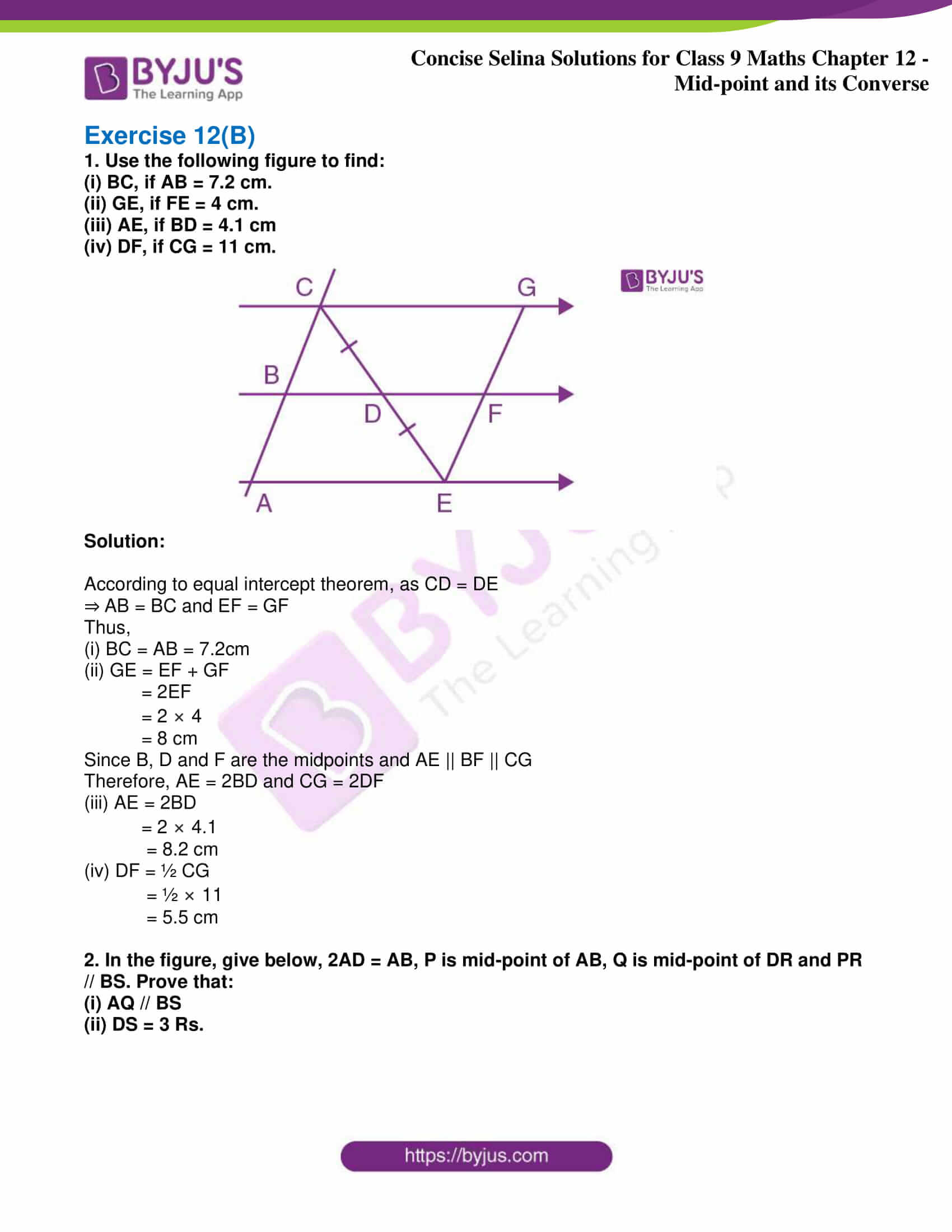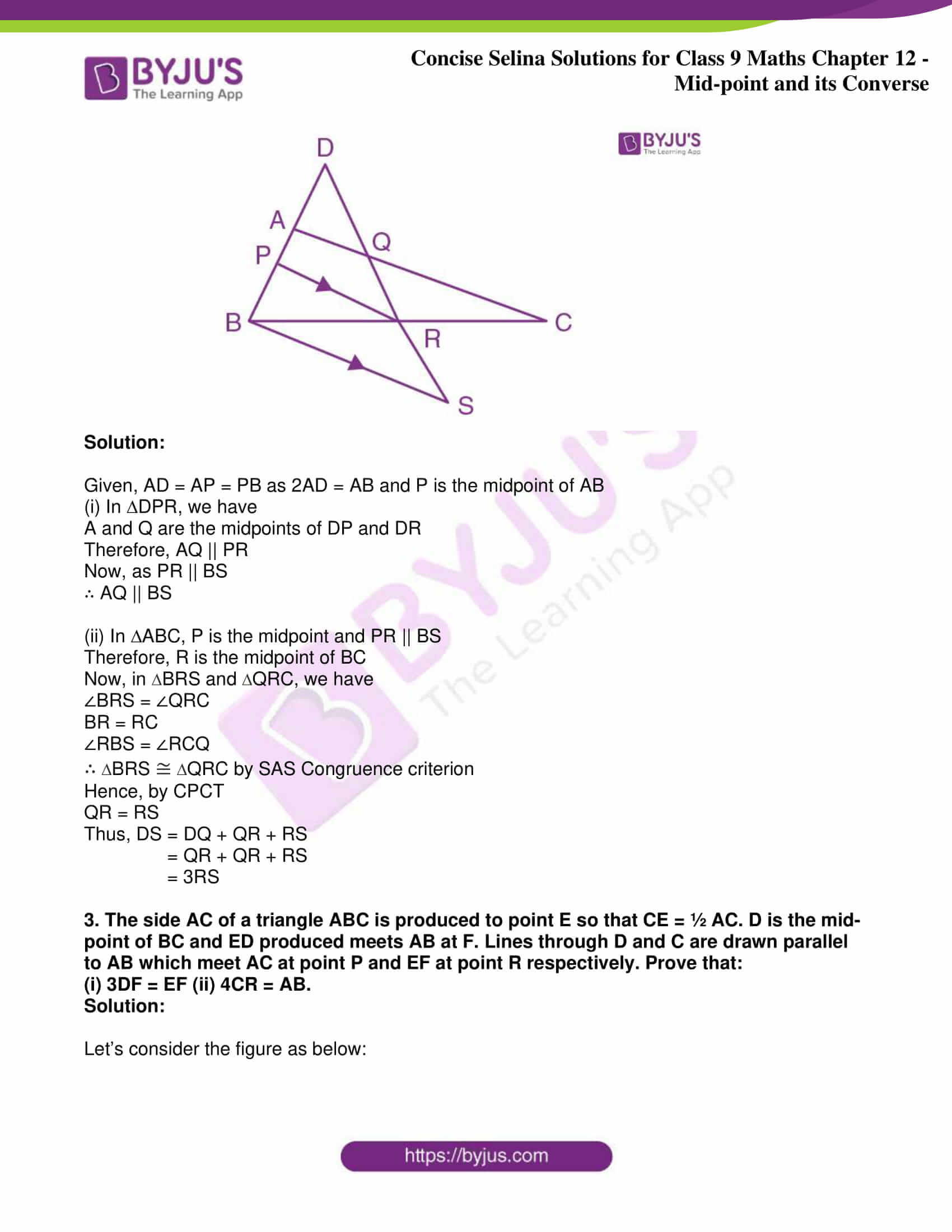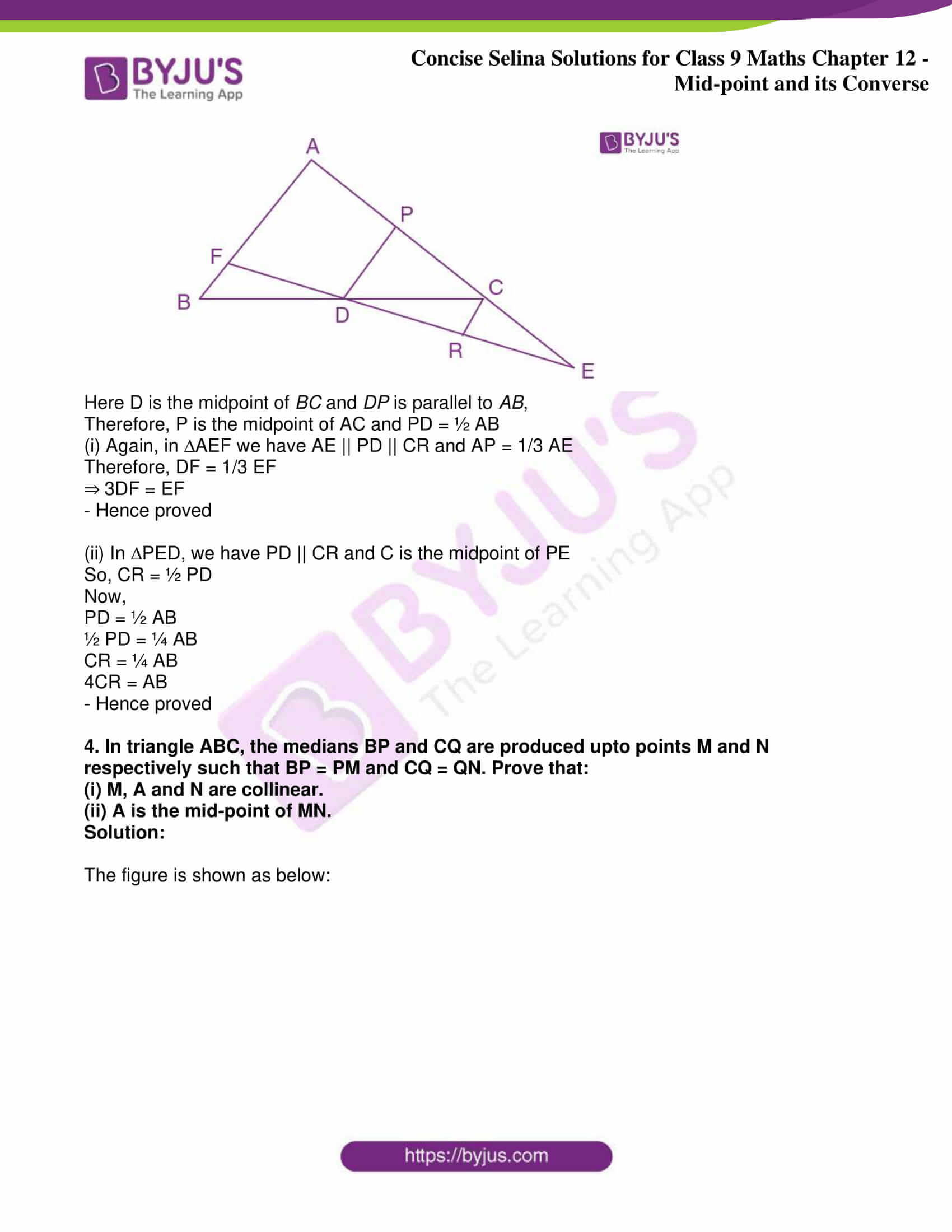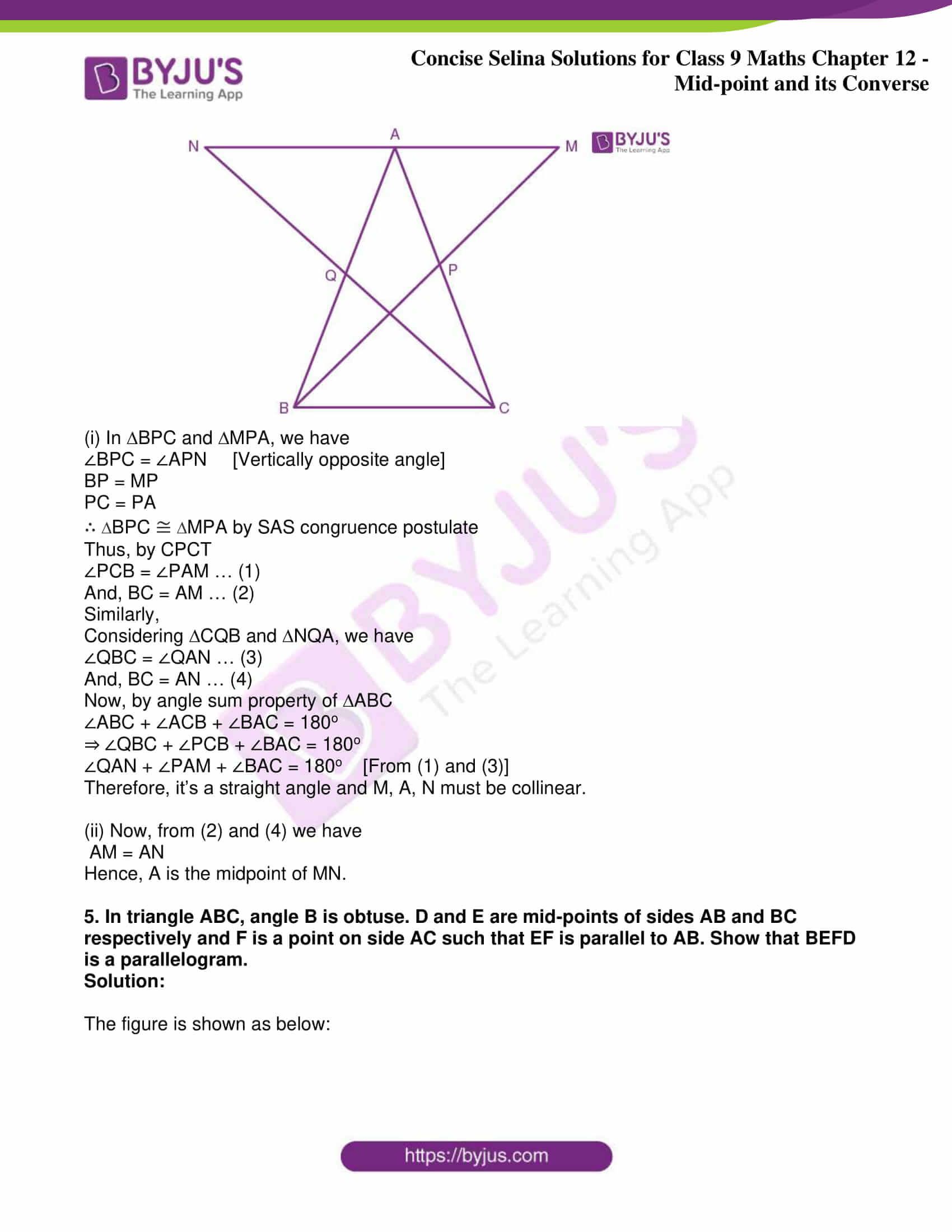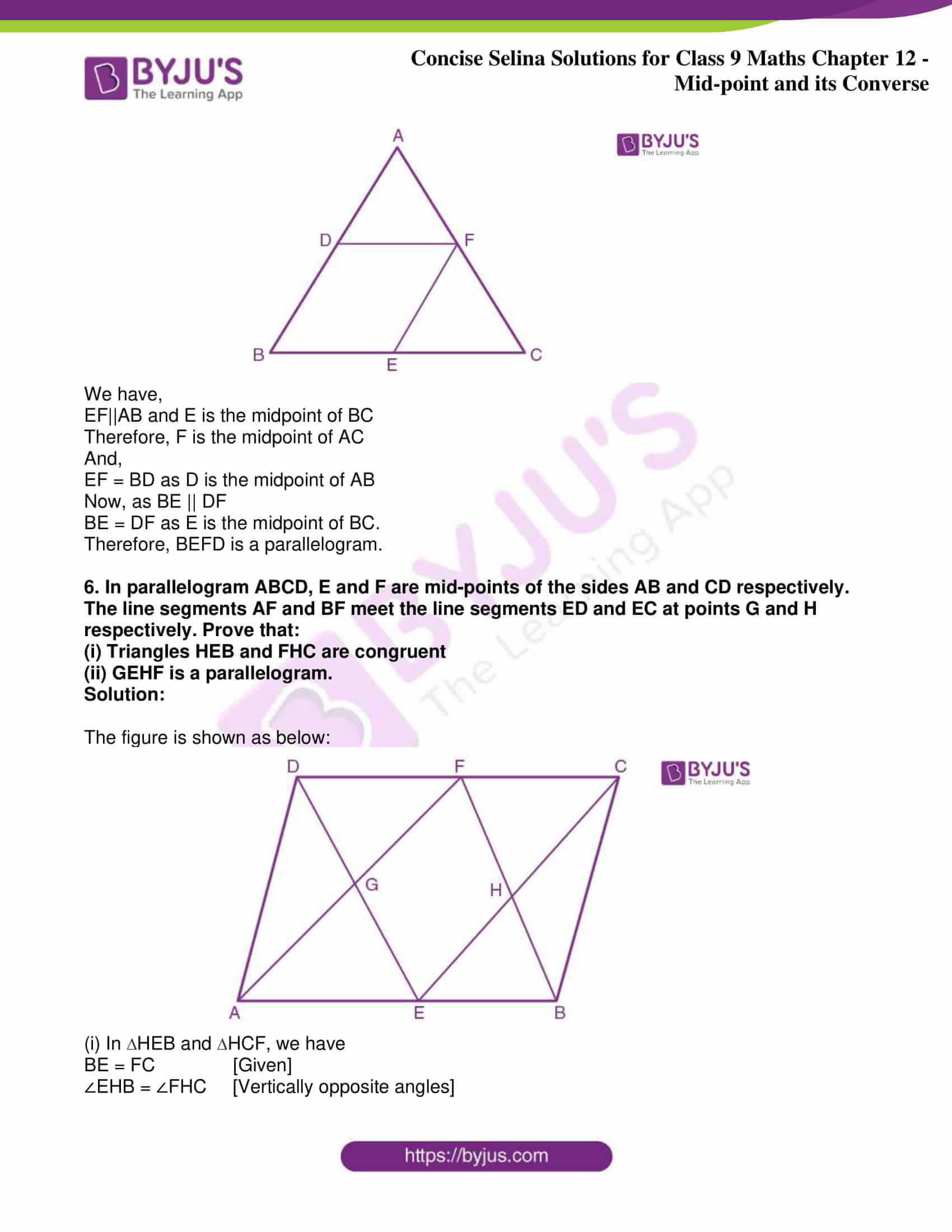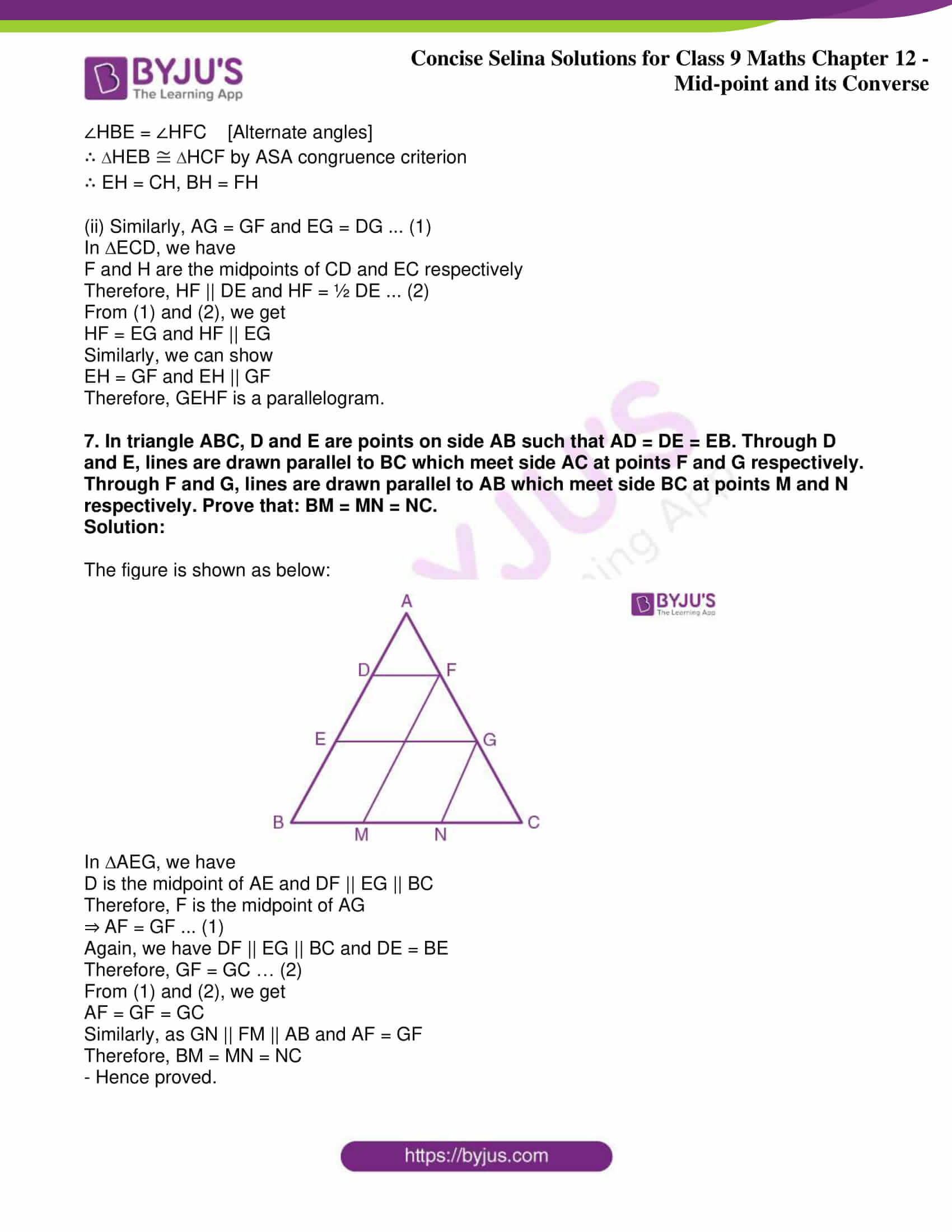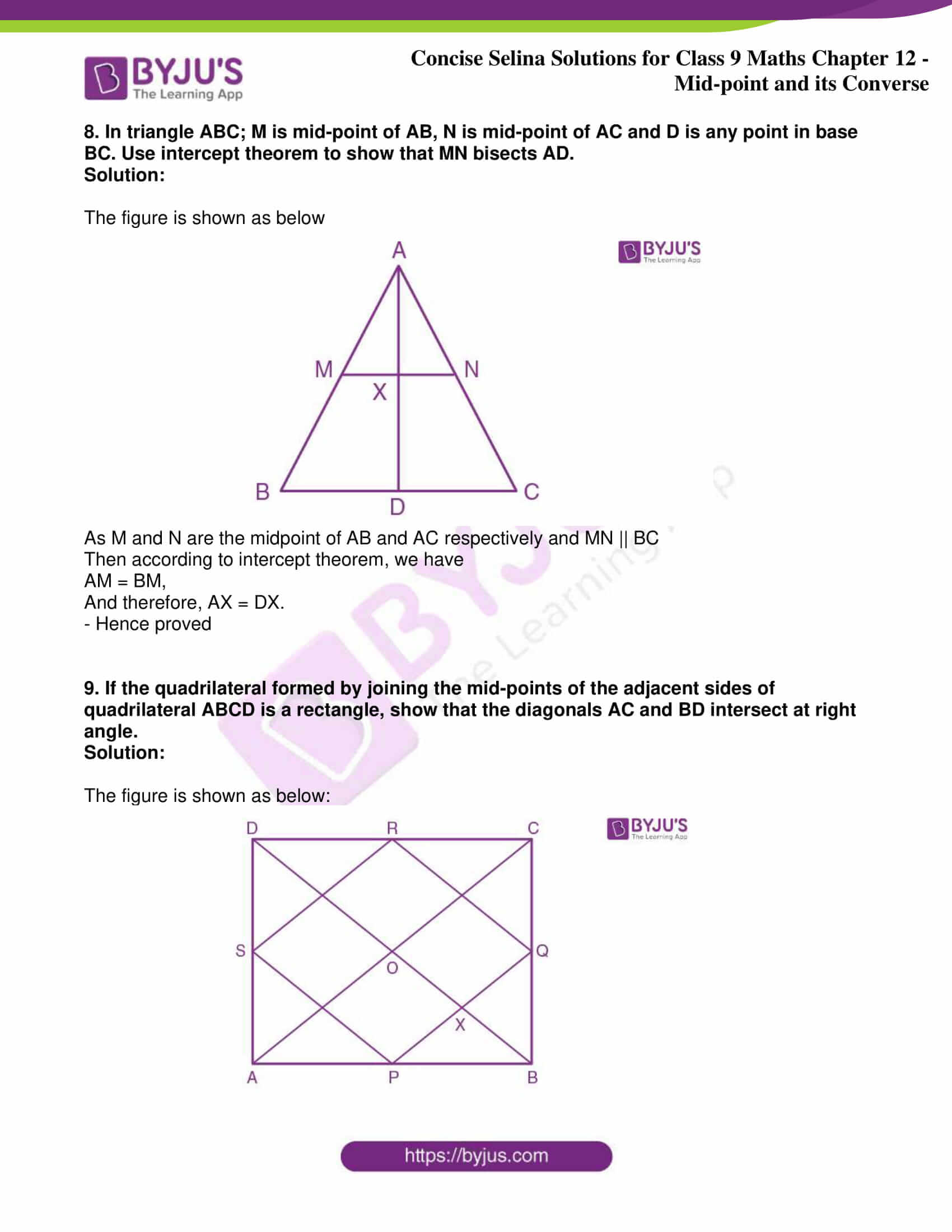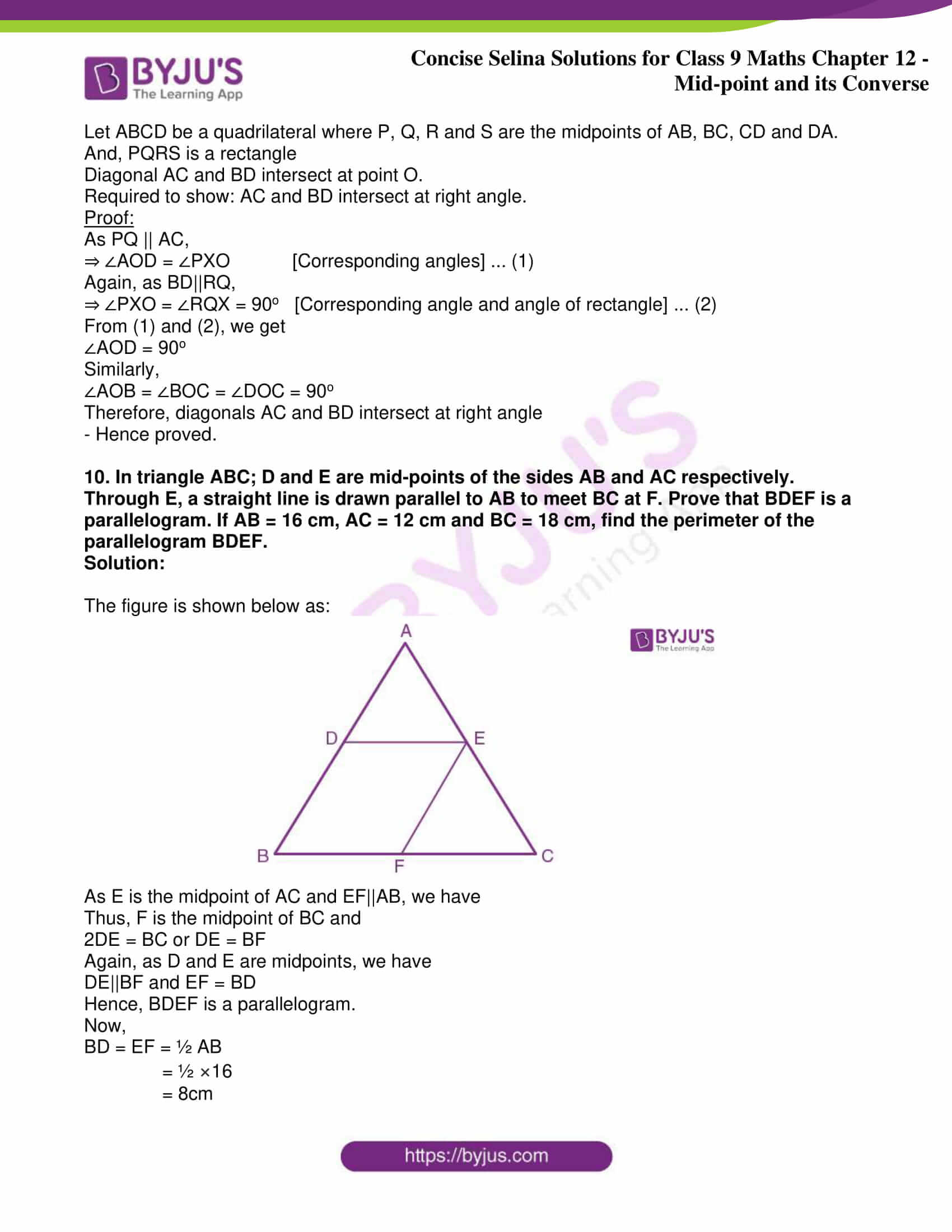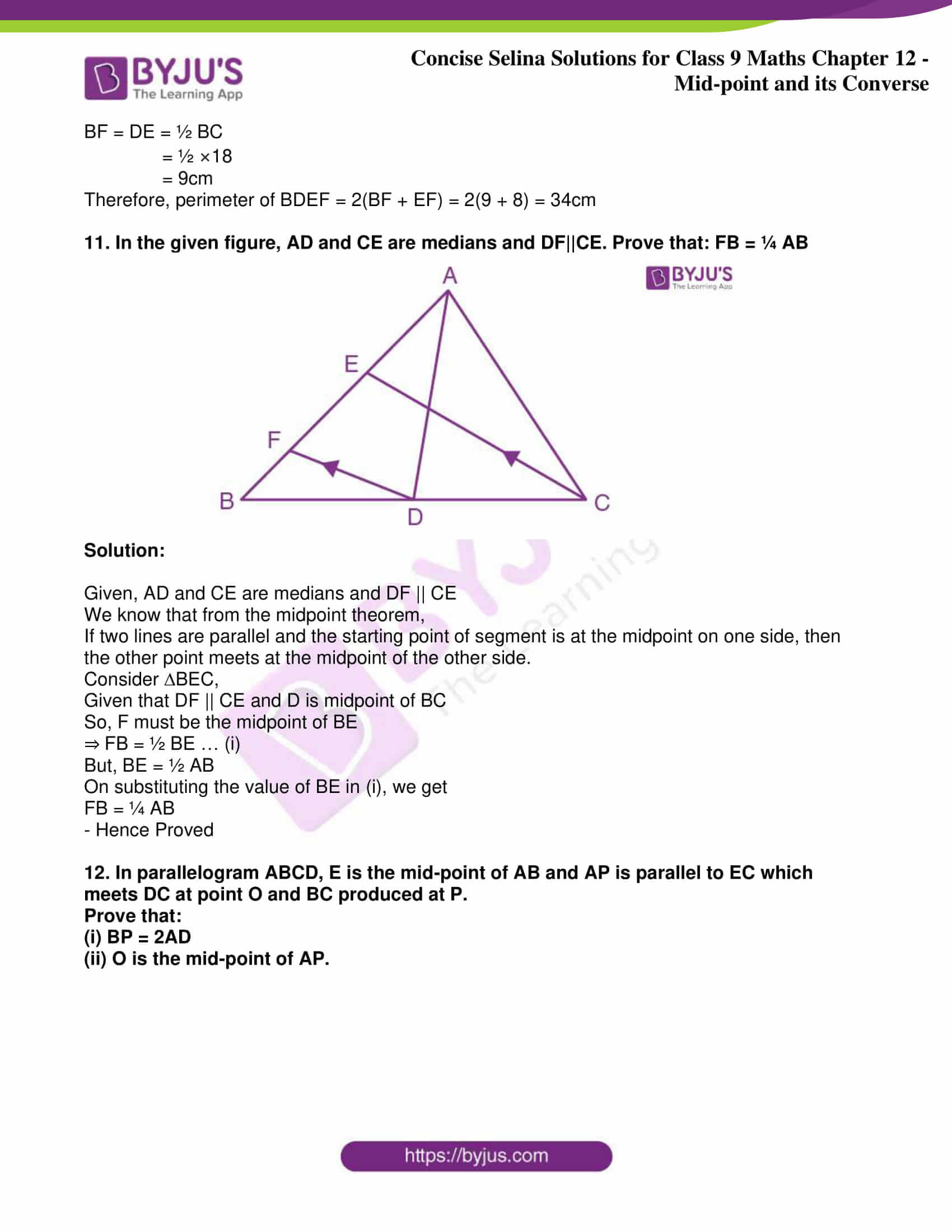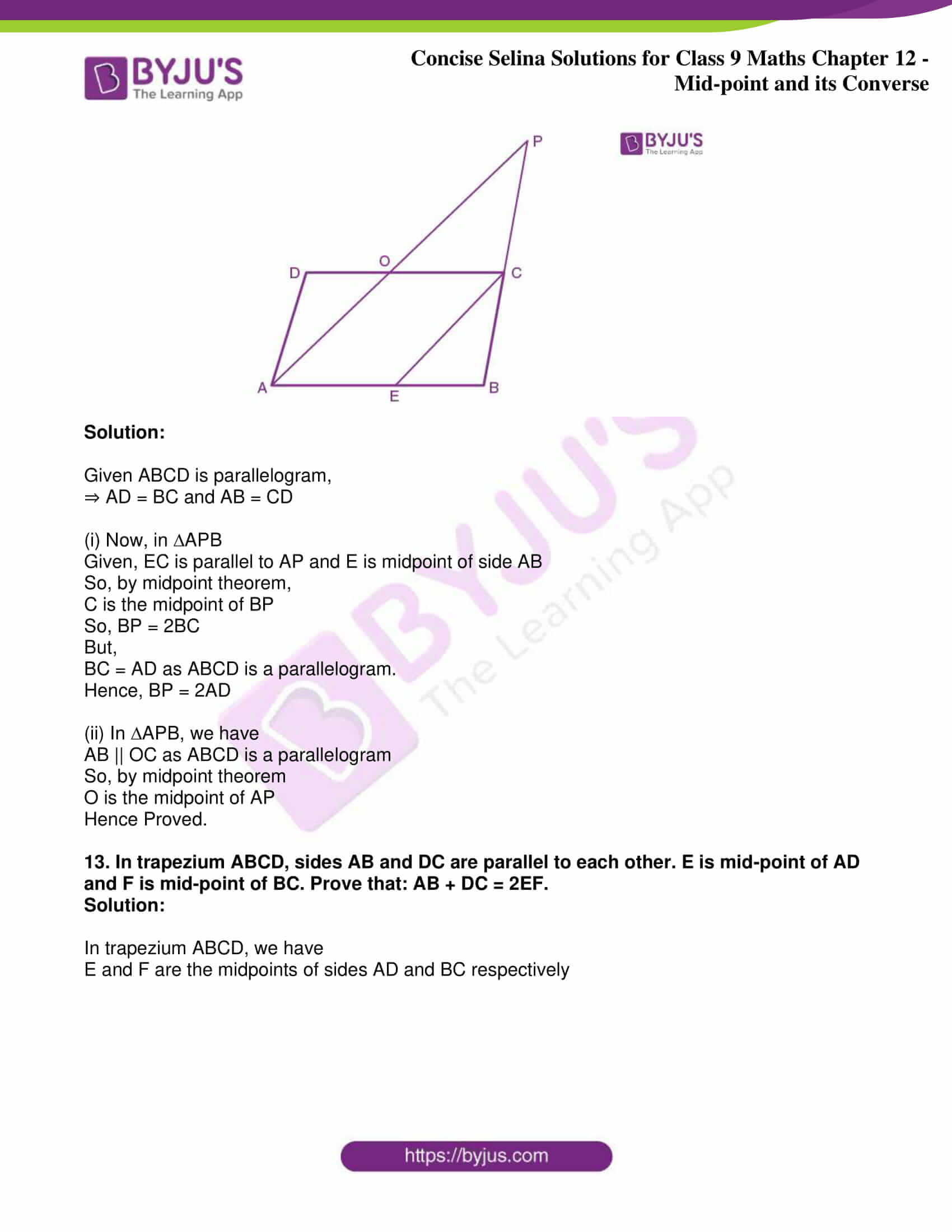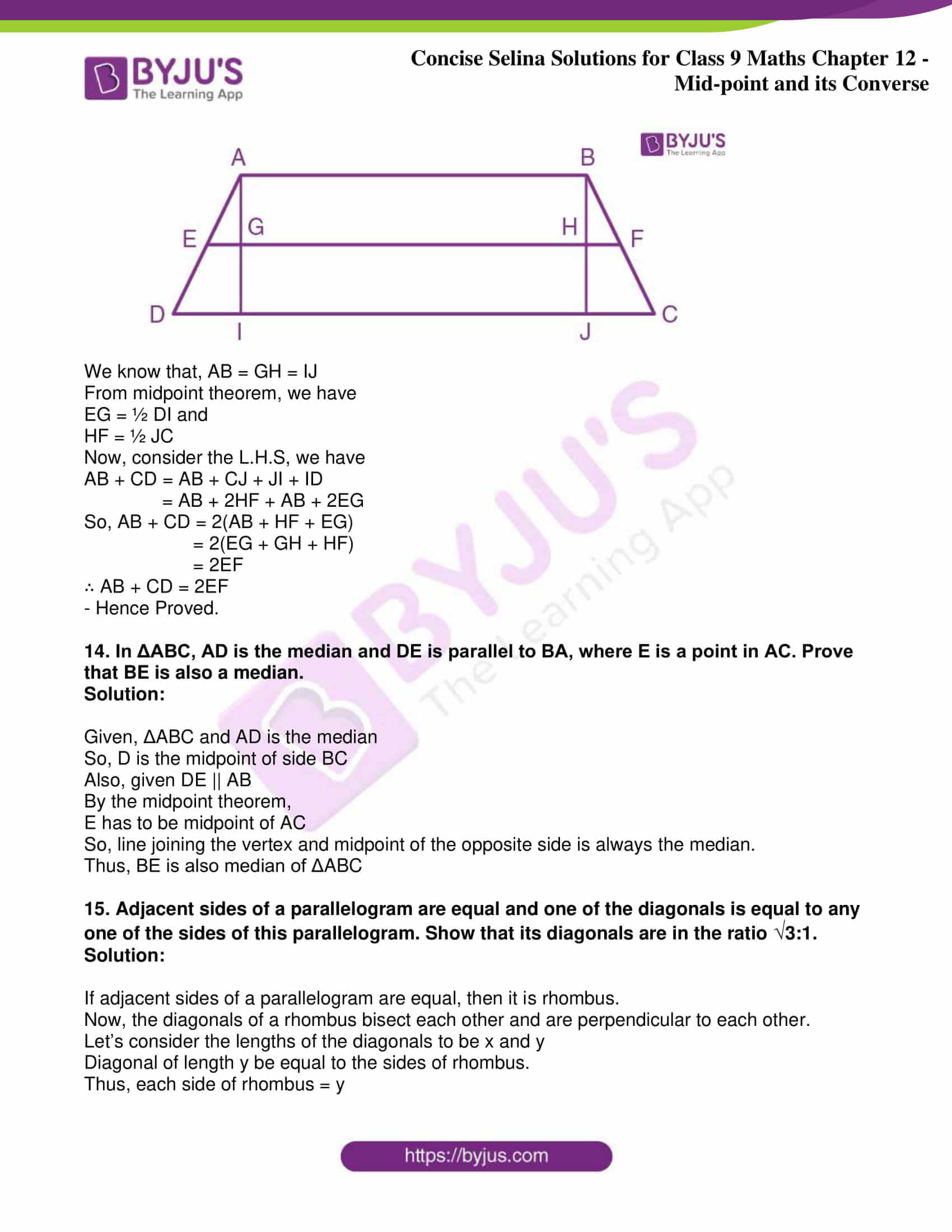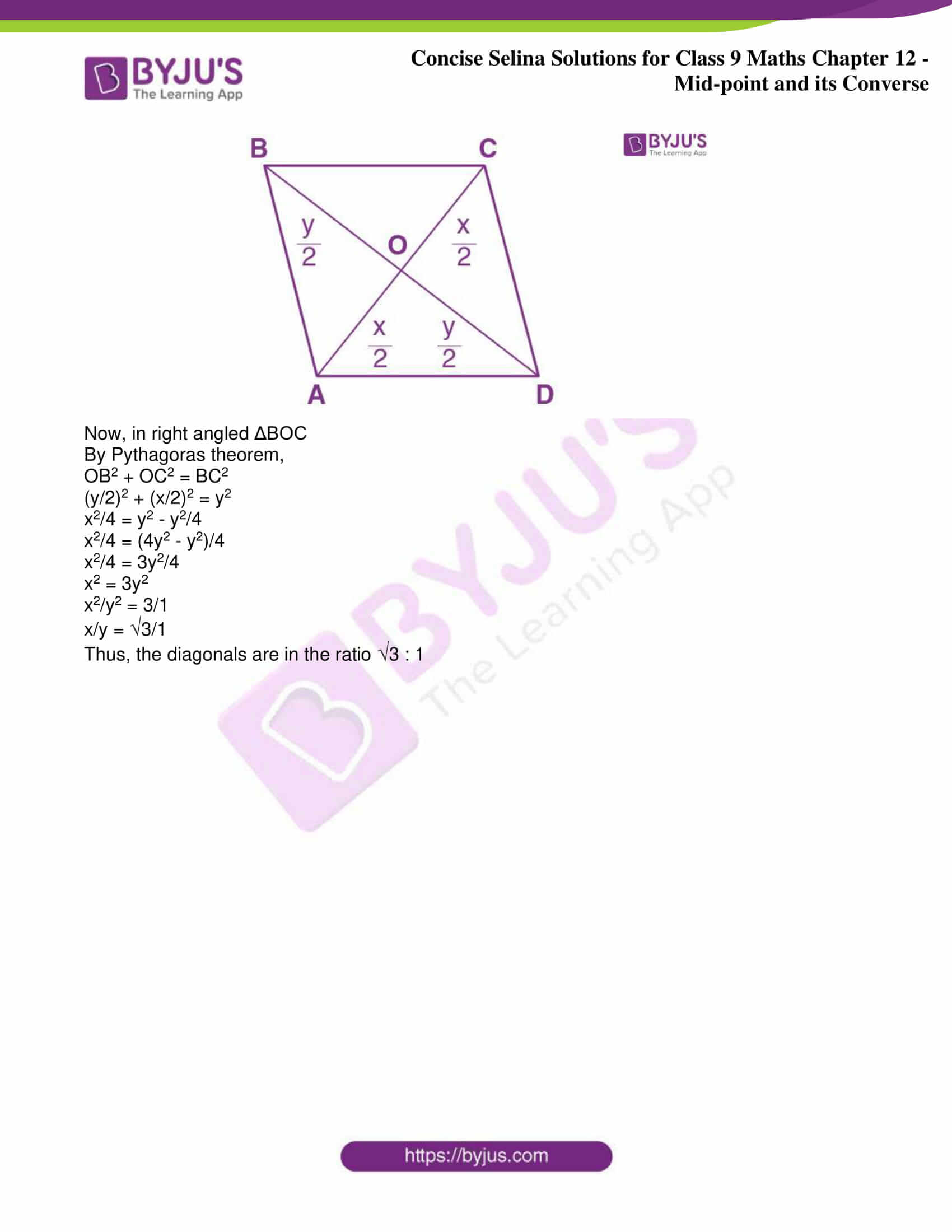Exercise 12(A)

1. In triangle ABC, M is mid-point of AB and a straight line through M and parallel to BC cuts AC in N. Find the lengths of AN and MN if BC = 7 cm and AC = 5 cm.

Solution:

The triangle is shown as below: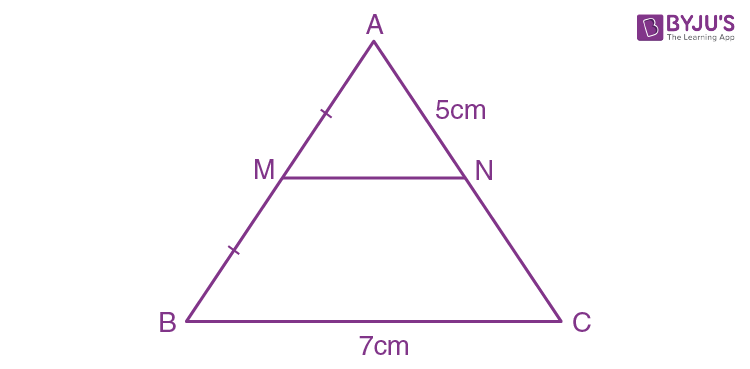Since M is the midpoint of AB and MN || BC

Then, by mid-point theorem N is the midpoint of AC.

Therefore,

MN = ½ BC = ½ × 7 = 3.5cm

And, AN = ½ AC = ½ × 5 = 2.5cm

2. Prove that the figure obtained by joining the mid-points of the adjacent sides of a rectangle is a rhombus.

Solution:

The figure is shown as below: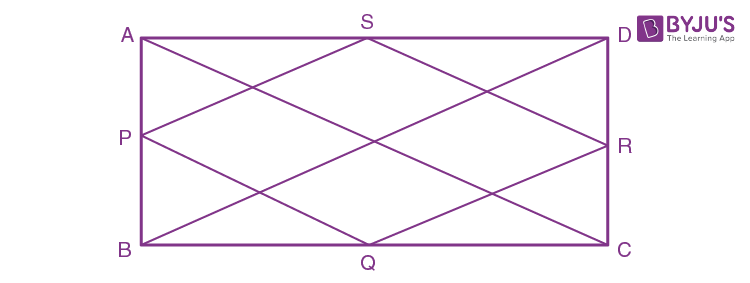Let ABCD be a rectangle where P, Q, R, S are the midpoint of AB, BC, CD, DA. Then, we need to show that PQRS is a rhombus.

Let’s draw two diagonals BD and AC as shown in figure

And, BD = AC [Since diagonals of rectangle are equal]

Proof:

From ∆ABD and ∆BCD, we have

PS = ½ BD = QR and PS || BD || QR

2PS = 2QR = BD and PS || QR … (1)

Similarly,

2PQ = 2SR = AC and PQ || SR … (2)

From (1) and (2) we get

PQ = QR = RS = PS

Therefore, PQRS is a rhombus.

– Hence proved

3. D, E and F are the mid-points of the sides AB, BC and CA of an isosceles ABC in which AB = BC. Prove that DEF is also isosceles.

Solution:

The figure is shown as below: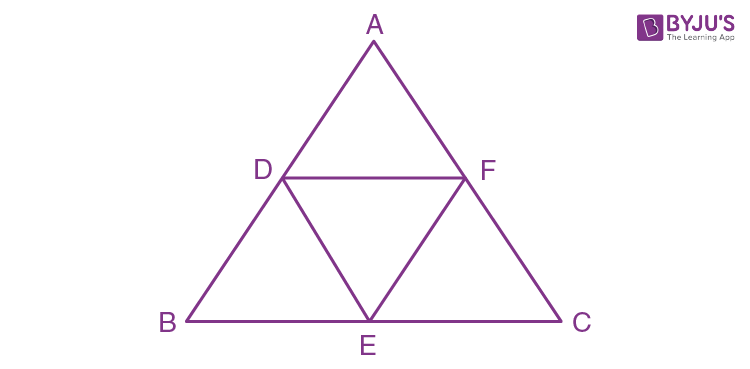Given, ABC is an isosceles triangle and AB = AC

Since D, E and F are midpoints of AB, BC and CA respectively

Therefore, by mid-point theorem

2DE = AC and 2EF = AB

⇒ DE = EF

Therefore, DEF is an isosceles triangle where DE = EF.

– Hence proved

4. The following figure shows a trapezium ABCD in which AB // DC. P is the mid-point of AD and PR // AB. Prove that:

PR = ½ (AB + CD)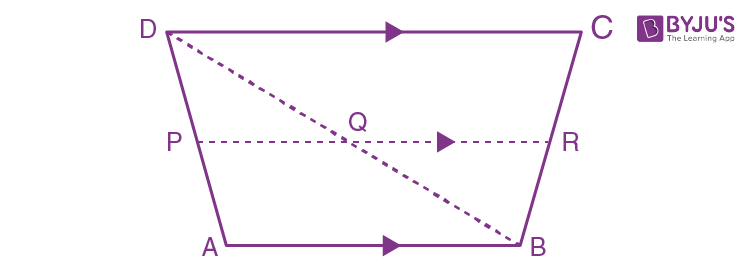Solution:

Given,

In ∆ABD, P is the midpoint of AD and PR || AB

Therefore, Q is the midpoint of BD [By mid-point theorem]

Similarly, R is the midpoint of BC as PR || CD || AB

Now, from ∆ABD

2PQ = AB … (1)

And, from ∆BCD

2QR = CD … (2)

Adding (1) and (2), we get

2(PQ + QR) = AB + CD

2PR = AB + CD

PR = ½ (AB + CD)

– Hence proved.

5. The figure, given below, shows a trapezium ABCD. M and N are the mid-point of the non-parallel sides AD and BC respectively. Find: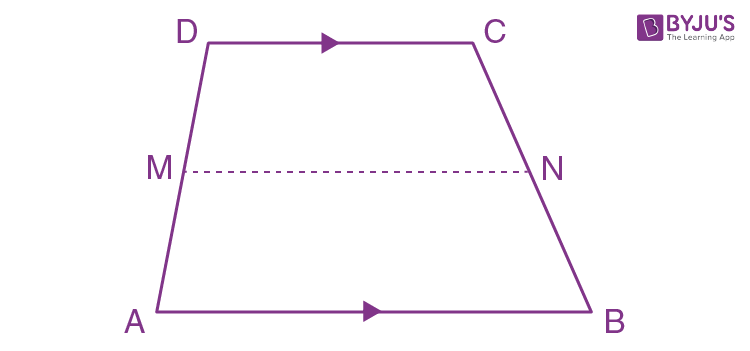(i) MN, if AB = 11 cm and DC = 8 cm.

(ii) AB, if DC = 20 cm and MN = 27 cm.

(iii) DC, if MN = 15 cm and AB = 23 cm.

Solution:

Let’s draw a diagonal AC as shown in the figure below,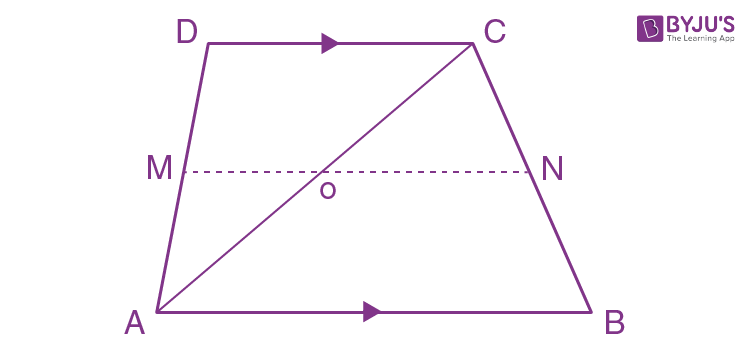(i) Given, AB = 11cm and CD = 8cm

From ∆ABC, we have

ON = ½ AB = ½ × 11 = 5.5cm

From ∆ACD, we have

OM = ½ CD = ½ × 8 = 4cm

Hence, MN = OM +ON

= (4 + 5.5)

= 9.5cm

(ii) Given, CD = 20cm and MN = 27cm

From ∆ACD, we have

OM = ½ CD = ½ × 20 = 10cm

Therefore, ON = 27 – 10 = 17cm

Then from ∆ABC, we have

AB = 2 ON

= 2 × 17

= 34cm

(iii) Given, AB = 23cm and MN = 15cm

From ∆ABC, we have

ON = ½ AB = ½ × 23 = 11.5cm

Therefore, OM = 15 – 11.5 = 3.5cm

Then from ∆ACD, we have

CD = 2 OM

= 2 × 3.5

= 7cm

6. The diagonals of a quadrilateral intersect at right angles. Prove that the figure obtained by joining the mid-points of the adjacent sides of the quadrilateral is rectangle.

Solution:

The figure is shown as below: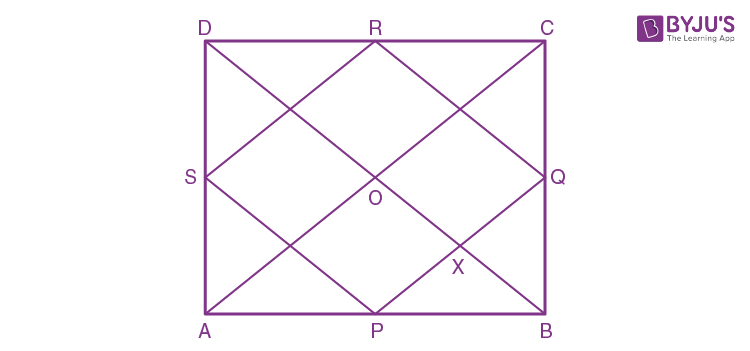Let ABCD be a quadrilateral where P, Q, R, S are the midpoints of AB, BC, CD, DA.

Diagonals AC and BD intersects at right angles at point O.

Required to prove: PQRS is a rectangle

Proof:

From ∆ABC and ∆ADC, we have

2PQ = AC and PQ || AC … (1)

2RS = AC and RS || AC … (2)

From (1) and (2) we get,

PQ = RS and PQ || RS

Similarly,

PS = RQ and PS||RQ

Therefore, PQRS is a parallelogram.

Now as PQ||AC, we have

∠AOD = ∠PXO = 90o [Corresponding angles]

Again, as BD||RQ, we have

∠PXO = ∠RQX = 90o [Corresponding angle]

Similarly,

∠QRS = ∠RSP = ∠SPQ = 90o

Therefore, PQRS is a rectangle.

– Hence proved

7. L and M are the mid-point of sides AB and DC respectively of parallelogram ABCD. Prove that segments DL and BM trisect diagonal AC.

Solution:

The required figure is shown as below: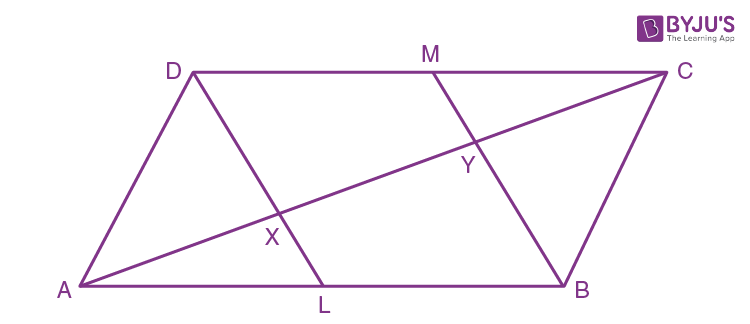We have,

BL = DM and BL || DM and BLMD is a parallelogram

⇒ BM || DL

Now, in ∆ABY, we have

L is the midpoint of AB and XL || BY,

Therefore, x is the midpoint of AY

⇒ AX = XY … (1)

Similarly for triangle CDX

⇒ CY = XY … (2)

From (1) and (2), we get

AX = XY = CY and AC = AX + XY + CY

Thus, segments DL and BM trisect the diagonal AC of parallelogram ABCD

– Hence proved

8. ABCD is a quadrilateral in which AD = BC. E, F, G and H are the mid-points of AB, BD, CD and Ac respectively. Prove that EFGH is a rhombus.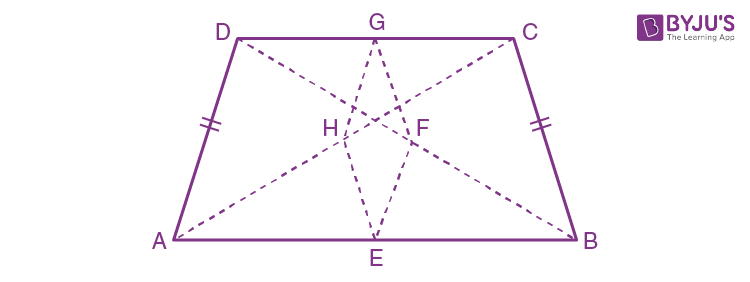Solution:

Given, AD = BC … (1)

From the figure,

In ∆ADC and ∆ABD, we have

⇒ 2GH = 2EF = AD … (2)

Now, in ∆BCD and ∆ABC, we have

2GF = BC and 2EH = BC

⇒ 2GF = 2EH = BC … (3)

From (1), (2), (3) we get

2GH = 2EF = 2GF = 2EH

⇒ GH = EF = GF = EH

Therefore, EFGH is a rhombus.

– Hence proved

9. A parallelogram ABCD has P the mid-point of DC and Q a point of AC such that CQ = ¼ AC. PQ produced meets BC at R.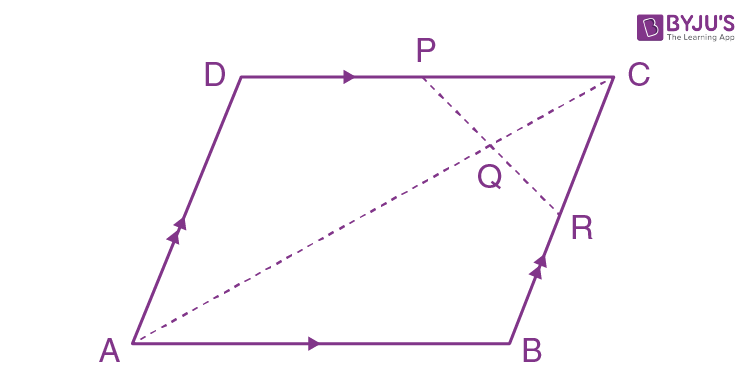Prove that:

(i) R is the midpoint of BC

(ii) PR = ½ DB

Solution:

Let’s draw the diagonal BD as shown below.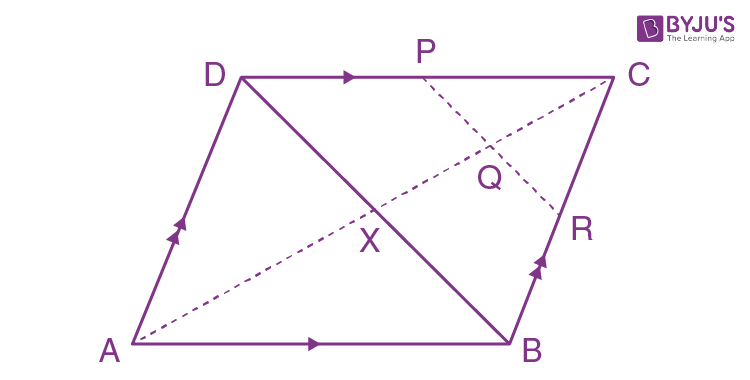The diagonal AC and BD cuts at point X.

We know that the diagonals of a parallelogram bisect each other.

Therefore, AX = CX and BX = DX

Given,

CQ = ¼ AC

CQ = ¼ × 2CX

CQ = ½ CX

Therefore, Q is the midpoint of CX.

(i) For ∆CDX, PQ || DX or PR || BD

And in ∆CBX, Q is the midpoint of CX and QR||BX

Therefore, R is the midpoint of BC

(ii) In ∆BCD,

As P and R are the midpoint of CD and B, we have

Thus, PR = ½ DB

10. D, E and F are the mid-points of the sides AB, BC and CA respectively of ABC. AE meets DF at O. P and Q are the mid-points of OB and OC respectively. Prove that DPQF is a parallelogram.

Solution:

The required figure is shown as below: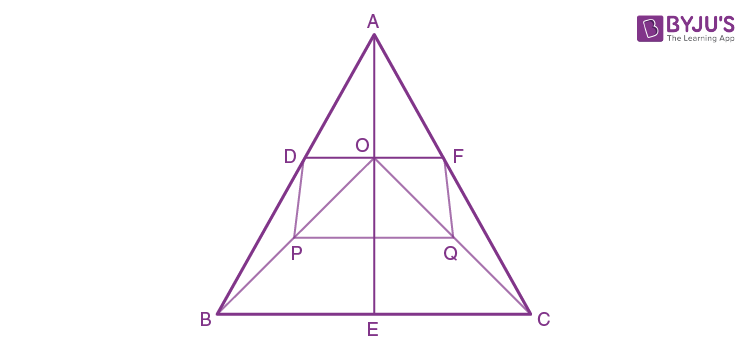In ∆ABC and ∆OBC, we have

2DE = BC and 2PQ = BC,

Therefore, DE = PQ … (1)

In ∆ABO and ∆ACO, we have

2PD = AO and 2FQ = AO,

Therefore, PD = FQ … (2)

From (1) and (2), we get that PQFD is a parallelogram.

– Hence proved.

11. In triangle ABC, P is the mid-point of side BC. A line through P and parallel to CA meets AB at point Q; and a line through Q and parallel to BC meets median AP at point R. Prove that:

(i) AP = 2AR

(ii) BC = 4QR

Solution:

The required figure is shown as below: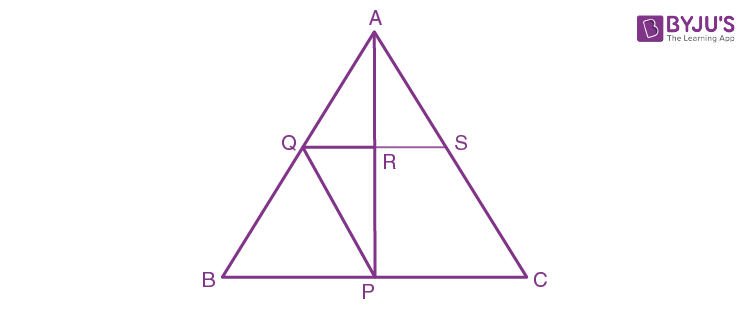It’s seen that P is the midpoint of BC, PQ || AC and QR || BC

Therefore, Q is the midpoint of AB and R is the midpoint of AP

(i) Thus, AP = 2AR

(ii) Let’s extend QR such that it cuts AC at S as shown in the figure.

Now, in ∆PQR and ∆ARS, we have

∠PQR = ∠ARS (Opposite angles)

PR = AR

PQ = AS (Since, PQ = AS = ½ AC)

Thus, ∆PQR ≅ ∆ARS by SAS congruence criterion

Therefore, by CPCT

QR = RS

Now,

BC = 2QS

BC = 2 × 2QR

BC = 4QR

– Hence proved

12. In trapezium ABCD, AB is parallel to DC; P and Q are the mid-points of AD and BC respectively. BP produced meets CD produced at point E. Prove that:

(i) Point P bisects BE,

(ii) PQ is parallel to AB.

Solution:

The required figure is shown as below: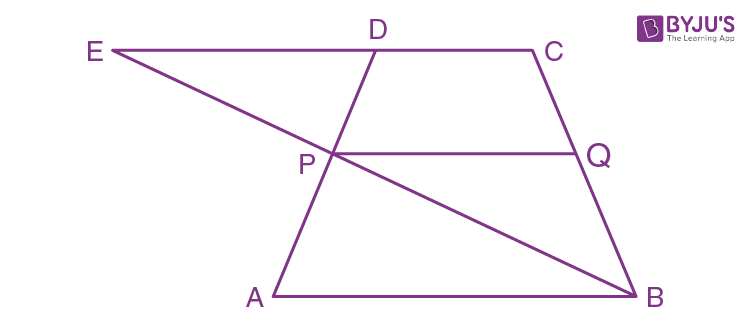(i) In ∆PED and ∆ABP, we have

PD = AP [Since, P is the mid-point of AD]

∠DPE = ∠APB [Opposite angles]

∠PED = ∠PBA [Alternate angles as AB || CE]

∴ ∆PED ≅ ∆ABP by ASA congruence postulate

Thus, by CPCT

EP = BP

(ii) For ∆ECB, we have PQ||CE

Also, CE||AB

Therefore, PQ||AB

– Hence proved

13. In a triangle ABC, AD is a median and E is mid-point of median AD. A line through B and E meets AC at point F. Prove that AC = 3AF.

Solution:

The required figure is shown as below: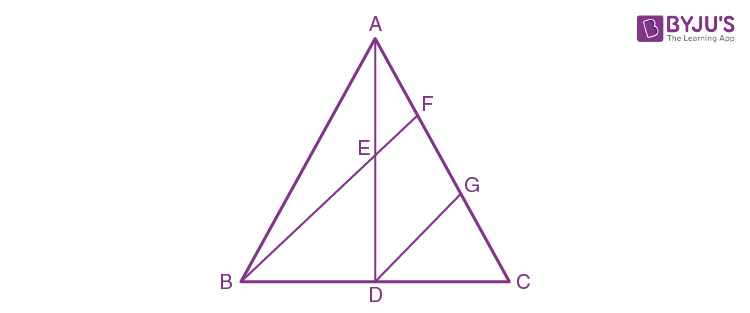Let’s draw a line DG || BF

Now,

DG || BF and E is the midpoint of AD

Therefore, F is the midpoint of AG

⇒ AF = GF … (1)

And, in ∆BCF, we have

DG || BF and D is the midpoint of BC

Therefore, G is the midpoint of CF

⇒ GF=CF … (2)

AC = AF + GF + CF [From figure]

AC = 3AF [From (1) and (2)]

– Hence proved.

14. D and F are mid-points of sides AB and AC of a triangle ABC. A line through F and parallel to AB meets BC at point E.

(i) Prove that BDFE is parallelogram

(ii) Find AB, if EF = 4.8 cm.

Solution:

The required figure is shown as below: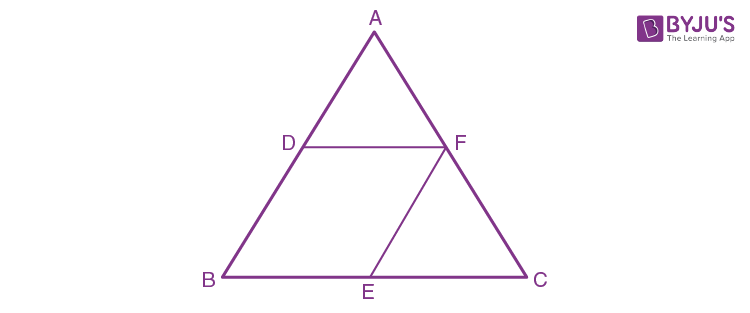(i) Since F is the midpoint and EF || AB

Therefore, E is the midpoint of BC

So, BE = ½ BC and EF = ½ AB … (1)

And,

Since D and F are the midpoint of AB and AC

Therefore, DE||BC

So, DF = ½ BC and DB = ½ AB … (2)

From (1) and (2), we get

BE = DF and BD = EF

Hence, BDEF is a parallelogram.

(ii) Now, AB = 2EF

= 2 × 4.8

= 9.6cm

15. In triangle ABC, AD is the median and DE, drawn parallel to side BA, meets AC at point E. Show that BE is also a median.

Solution: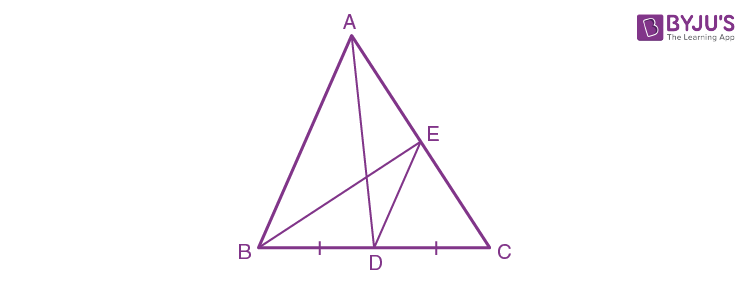In ∆ABC, we have

AD is the median of BC

D is the mid-point of BC

Given that DE || BA

So, by the converse of the mid-point theorem,

DE bisects AC

⇒ E is the mid-Point of AC

And, BE is the median of AC

Hence, BE is also a median.

16. In ABC, E is mid-point of the median AD and BE produced meets side AC at point Q. Show that BE: EQ = 3:1.

Solution:

Construction: Draw DY || BQ

In ∆BCQ and ∆DCY, we have

∠BCQ = DCY [Common]

∠BQC = ∠DYC [Corresponding angles]

So, ∆BCQ ~ ∆DCY by AA similarity criterion

Thus,

BQ/DY = BC/DC = CQ/CY [Corresponding sides are proportional]

BQ/DY = 2 … (i)

EQ/DY = AE/ED = ½ [E is the mid-point of AD]

⇒ EQ/DY = ½ … (ii)

On dividing (i) by(ii), we get

BQ/EQ = 4

BQ = 4 EQ

BE + EQ = 4EQ

BE = 3EQ

Therefore, BE/EQ = 3/1

17. In the given figure, M is mid-point of AB and DE, whereas N is mid-point of BC and DF. Show that: EF = AC.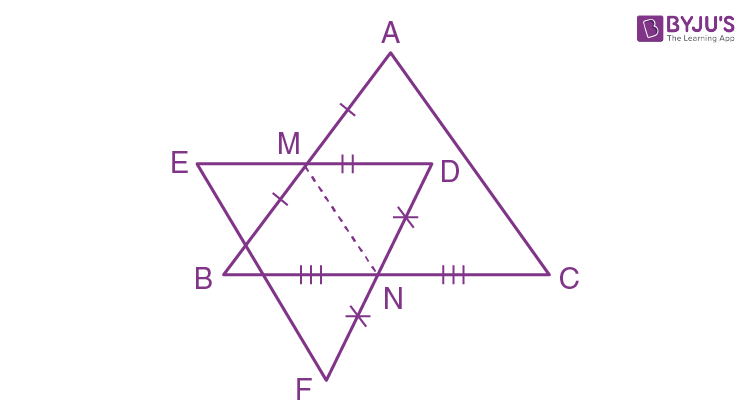Solution:

In ∆EDF, we have

M is the mid-point of AB and N is the mid-point of DE

So, MN = ½ EF (By mid-point theorem)

EF = 2MN … (i)

In ∆ABC, we have

M is the mid-point of AB and N is the mid-point of BC

MN = ½ AC (By mid-point theorem)

AC = 2MN … (ii)

From (i) and (ii), we get

EF = AC

Exercise 12(B)

1. Use the following figure to find:

(i) BC, if AB = 7.2 cm.

(ii) GE, if FE = 4 cm.

(iii) AE, if BD = 4.1 cm

(iv) DF, if CG = 11 cm.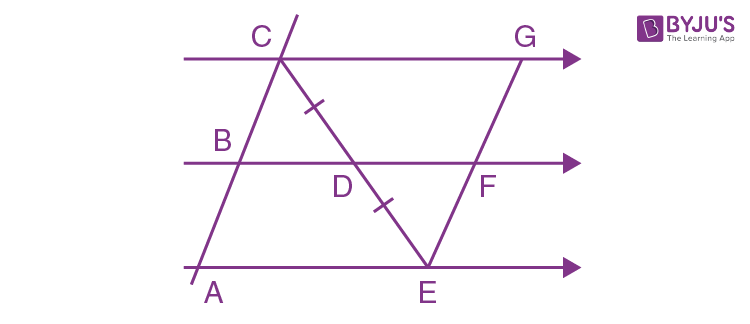Solution:

According to equal intercept theorem, as CD = DE

⇒ AB = BC and EF = GF

Thus,

(i) BC = AB = 7.2cm

(ii) GE = EF + GF

= 2EF

= 2 × 4

= 8 cm

Since B, D and F are the midpoints and AE || BF || CG

Therefore, AE = 2BD and CG = 2DF

(iii) AE = 2BD

= 2 × 4.1

= 8.2 cm

(iv) DF = ½ CG

= ½ × 11

= 5.5 cm

2. In the figure, give below, 2AD = AB, P is mid-point of AB, Q is mid-point of DR and PR // BS. Prove that:

(i) AQ // BS

(ii) DS = 3 Rs.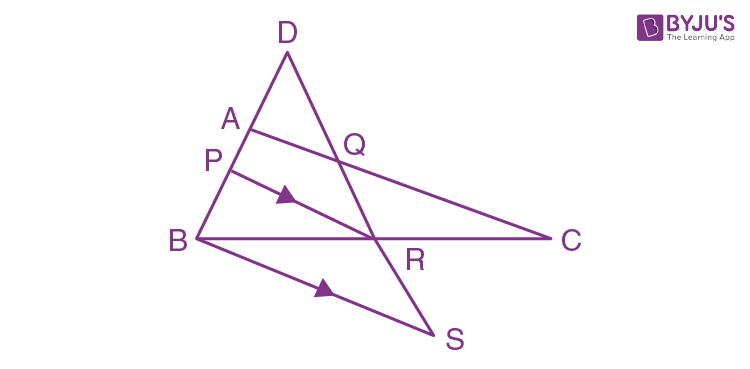Solution:

Given, AD = AP = PB as 2AD = AB and P is the midpoint of AB

(i) In ∆DPR, we have

A and Q are the midpoints of DP and DR

Therefore, AQ || PR

Now, as PR || BS

∴ AQ || BS

(ii) In ∆ABC, P is the midpoint and PR || BS

Therefore, R is the midpoint of BC

Now, in ∆BRS and ∆QRC, we have

∠BRS = ∠QRC

BR = RC

∠RBS = ∠RCQ

∴ ∆BRS ≅ ∆QRC by SAS Congruence criterion

Hence, by CPCT

QR = RS

Thus, DS = DQ + QR + RS

= QR + QR + RS

= 3RS

3. The side AC of a triangle ABC is produced to point E so that CE = ½ AC. D is the mid-point of BC and ED produced meets AB at F. Lines through D and C are drawn parallel to AB which meet AC at point P and EF at point R respectively. Prove that:

(i) 3DF = EF (ii) 4CR = AB.

Solution:

Let’s consider the figure as below: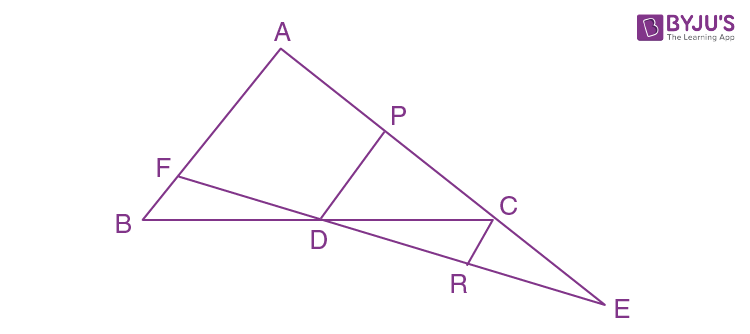Here D is the midpoint of BC and DP is parallel to AB,

Therefore, P is the midpoint of AC and PD = ½ AB

(i) Again, in ∆AEF we have AE || PD || CR and AP = 1/3 AE

Therefore, DF = 1/3 EF

⇒ 3DF = EF

– Hence proved

(ii) In ∆PED, we have PD || CR and C is the midpoint of PE

So, CR = ½ PD

Now,

PD = ½ AB

½ PD = ¼ AB

CR = ¼ AB

4CR = AB

– Hence proved

4. In triangle ABC, the medians BP and CQ are produced upto points M and N respectively such that BP = PM and CQ = QN. Prove that:

(i) M, A and N are collinear.

(ii) A is the mid-point of MN.

Solution:

The figure is shown as below: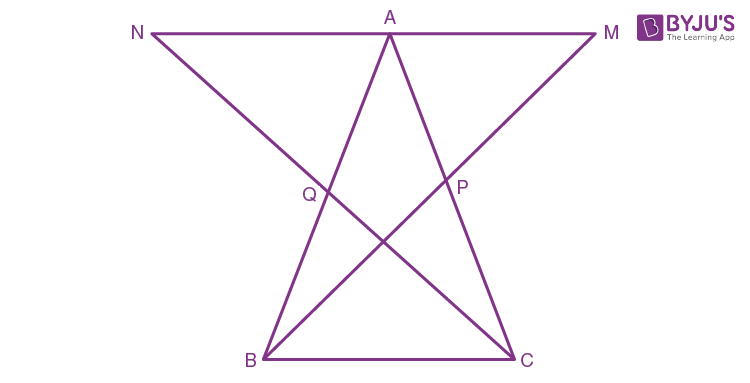(i) In ∆BPC and ∆MPA, we have

∠BPC = ∠APN [Vertically opposite angle]

BP = MP

PC = PA

∴ ∆BPC ≅ ∆MPA by SAS congruence postulate

Thus, by CPCT

∠PCB = ∠PAM … (1)

And, BC = AM … (2)

Similarly,

Considering ∆CQB and ∆NQA, we have

∠QBC = ∠QAN … (3)

And, BC = AN … (4)

Now, by angle sum property of ∆ABC

∠ABC + ∠ACB + ∠BAC = 180o

⇒ ∠QBC + ∠PCB + ∠BAC = 180o

∠QAN + ∠PAM + ∠BAC = 180o [From (1) and (3)]

Therefore, it’s a straight angle and M, A, N must be collinear.

(ii) Now, from (2) and (4) we have

AM = AN

Hence, A is the midpoint of MN.

5. In triangle ABC, angle B is obtuse. D and E are mid-points of sides AB and BC respectively and F is a point on side AC such that EF is parallel to AB. Show that BEFD is a parallelogram.

Solution:

The figure is shown as below:We have,

EF||AB and E is the midpoint of BC

Therefore, F is the midpoint of AC

And,

EF = BD as D is the midpoint of AB

Now, as BE || DF

BE = DF as E is the midpoint of BC.

Therefore, BEFD is a parallelogram.

6. In parallelogram ABCD, E and F are mid-points of the sides AB and CD respectively. The line segments AF and BF meet the line segments ED and EC at points G and H respectively. Prove that:

(i) Triangles HEB and FHC are congruent

(ii) GEHF is a parallelogram.

Solution:

The figure is shown as below: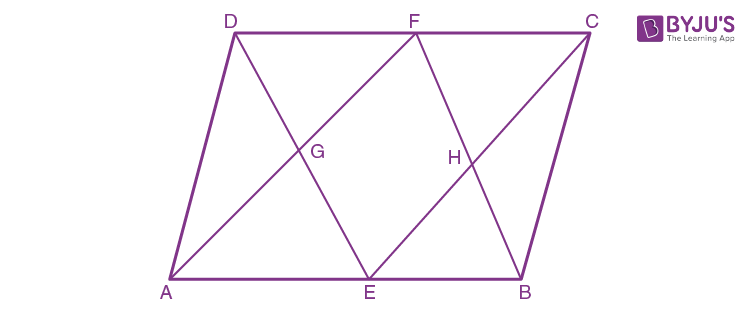(i) In ∆HEB and ∆HCF, we have

BE = FC [Given]

∠EHB = ∠FHC [Vertically opposite angles]

∠HBE = ∠HFC [Alternate angles]

∴ ∆HEB ≅ ∆HCF by ASA congruence criterion

∴ EH = CH, BH = FH

(ii) Similarly, AG = GF and EG = DG … (1)

In ∆ECD, we have

F and H are the midpoints of CD and EC respectively

Therefore, HF || DE and HF = ½ DE … (2)

From (1) and (2), we get

HF = EG and HF || EG

Similarly, we can show

EH = GF and EH || GF

Therefore, GEHF is a parallelogram.

7. In triangle ABC, D and E are points on side AB such that AD = DE = EB. Through D and E, lines are drawn parallel to BC which meet side AC at points F and G respectively. Through F and G, lines are drawn parallel to AB which meet side BC at points M and N respectively. Prove that: BM = MN = NC.

Solution:

The figure is shown as below: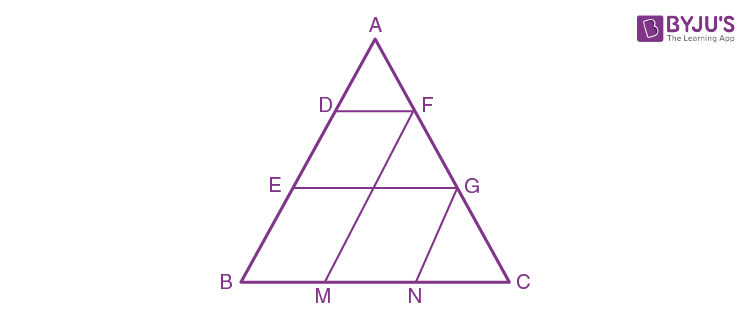In ∆AEG, we have

D is the midpoint of AE and DF || EG || BC

Therefore, F is the midpoint of AG

⇒ AF = GF … (1)

Again, we have DF || EG || BC and DE = BE

Therefore, GF = GC … (2)

From (1) and (2), we get

AF = GF = GC

Similarly, as GN || FM || AB and AF = GF

Therefore, BM = MN = NC

– Hence proved.

8. In triangle ABC; M is mid-point of AB, N is mid-point of AC and D is any point in base BC. Use intercept theorem to show that MN bisects AD.

Solution:

The figure is shown as below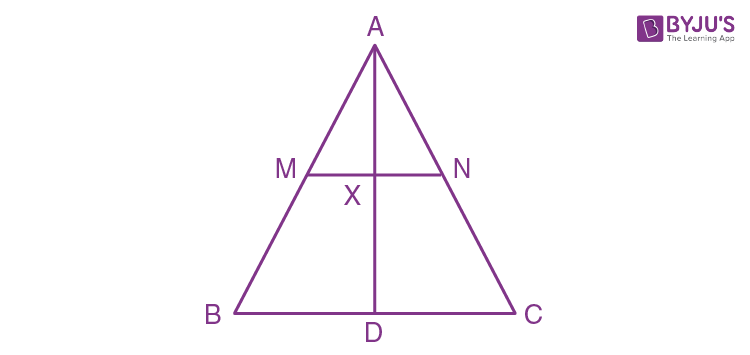As M and N are the midpoint of AB and AC respectively and MN || BC

Then according to intercept theorem, we have

AM = BM,

And therefore, AX = DX.

– Hence proved

9. If the quadrilateral formed by joining the mid-points of the adjacent sides of quadrilateral ABCD is a rectangle, show that the diagonals AC and BD intersect at right angle.

Solution:

The figure is shown as below: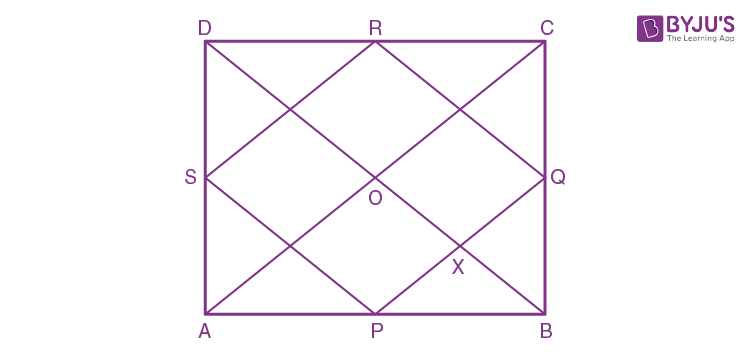Let ABCD be a quadrilateral where P, Q, R and S are the midpoints of AB, BC, CD and DA.

And, PQRS is a rectangle

Diagonal AC and BD intersect at point O.

Required to show: AC and BD intersect at right angle.

Proof:

As PQ || AC,

⇒ ∠AOD = ∠PXO [Corresponding angles] … (1)

Again, as BD||RQ,

⇒ ∠PXO = ∠RQX = 90o [Corresponding angle and angle of rectangle] … (2)

From (1) and (2), we get

∠AOD = 90o

Similarly,

∠AOB = ∠BOC = ∠DOC = 90o

Therefore, diagonals AC and BD intersect at right angle

– Hence proved.

10. In triangle ABC; D and E are mid-points of the sides AB and AC respectively. Through E, a straight line is drawn parallel to AB to meet BC at F. Prove that BDEF is a parallelogram. If AB = 16 cm, AC = 12 cm and BC = 18 cm, find the perimeter of the parallelogram BDEF.

Solution:

The figure is shown below as: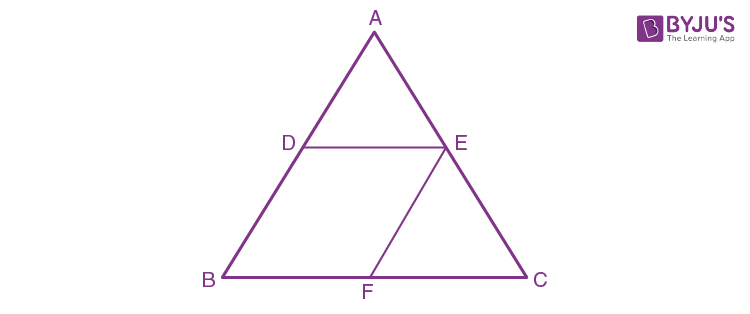As E is the midpoint of AC and EF||AB, we have

Thus, F is the midpoint of BC and

2DE = BC or DE = BF

Again, as D and E are midpoints, we have

DE||BF and EF = BD

Hence, BDEF is a parallelogram.

Now,

BD = EF = ½ AB

= ½ ×16

= 8cm

BF = DE = ½ BC

= ½ ×18

= 9cm

Therefore, perimeter of BDEF = 2(BF + EF) = 2(9 + 8) = 34cm

11. In the given figure, AD and CE are medians and DF||CE. Prove that: FB = ¼ AB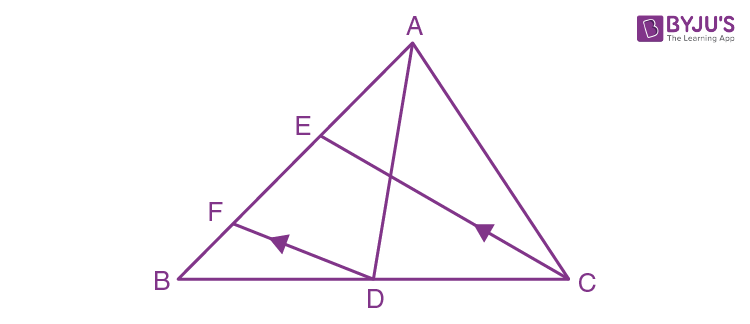Solution:

Given, AD and CE are medians and DF || CE

We know that from the midpoint theorem,

If two lines are parallel and the starting point of segment is at the midpoint on one side, then the other point meets at the midpoint of the other side.

Consider ∆BEC,

Given that DF || CE and D is midpoint of BC

So, F must be the midpoint of BE

⇒ FB = ½ BE … (i)

But, BE = ½ AB

On substituting the value of BE in (i), we get

FB = ¼ AB

– Hence Proved

12. In parallelogram ABCD, E is the mid-point of AB and AP is parallel to EC which meets DC at point O and BC produced at P.

Prove that:

(ii) O is the mid-point of AP.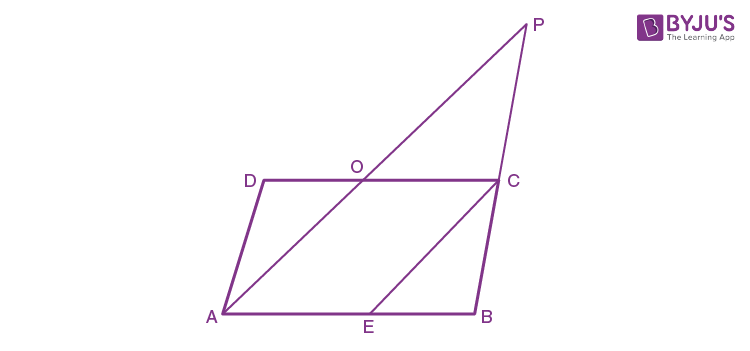Solution:

Given ABCD is parallelogram,

⇒ AD = BC and AB = CD

(i) Now, in ∆APB

Given, EC is parallel to AP and E is midpoint of side AB

So, by midpoint theorem,

C is the midpoint of BP

So, BP = 2BC

But,

BC = AD as ABCD is a parallelogram.

(ii) In ∆APB, we have

AB || OC as ABCD is a parallelogram

So, by midpoint theorem

O is the midpoint of AP

Hence Proved.

13. In trapezium ABCD, sides AB and DC are parallel to each other. E is mid-point of AD and F is mid-point of BC. Prove that: AB + DC = 2EF.

Solution:

In trapezium ABCD, we have

E and F are the midpoints of sides AD and BC respectively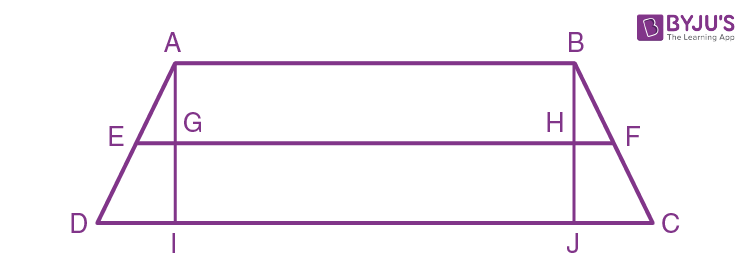We know that, AB = GH = IJ

From midpoint theorem, we have

EG = ½ DI and

HF = ½ JC

Now, consider the L.H.S, we have

AB + CD = AB + CJ + JI + ID

= AB + 2HF + AB + 2EG

So, AB + CD = 2(AB + HF + EG)

= 2(EG + GH + HF)

= 2EF

∴ AB + CD = 2EF

– Hence Proved.

14. In ΔABC, AD is the median and DE is parallel to BA, where E is a point in AC. Prove that BE is also a median.

Solution:

Given, ΔABC and AD is the median

So, D is the midpoint of side BC

Also, given DE || AB

By the midpoint theorem,

E has to be midpoint of AC

So, line joining the vertex and midpoint of the opposite side is always the median.

Thus, BE is also median of ΔABC

15. Adjacent sides of a parallelogram are equal and one of the diagonals is equal to any one of the sides of this parallelogram. Show that its diagonals are in the ratio 3:1.

Solution:

If adjacent sides of a parallelogram are equal, then it is rhombus.

Now, the diagonals of a rhombus bisect each other and are perpendicular to each other.

Let’s consider the lengths of the diagonals to be x and y

Diagonal of length y be equal to the sides of rhombus.

Thus, each side of rhombus = y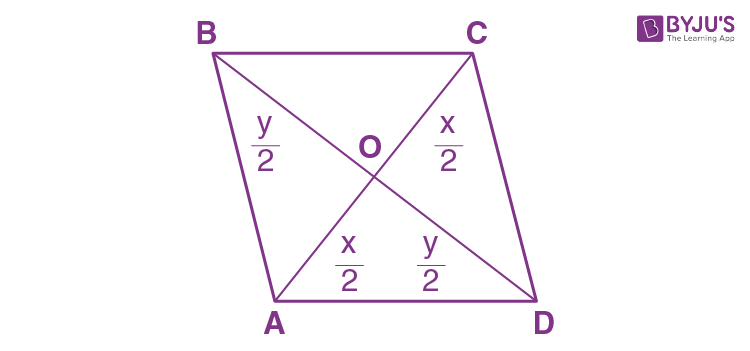Now, in right angled ΔBOC

By Pythagoras theorem,

OB2 + OC2 = BC2

(y/2)2 + (x/2)2 = y2

x2/4 = y2 – y2/4

x2/4 = (4y2 – y2)/4

x2/4 = 3y2/4

x2 = 3y2

x2/y2 = 3/1

x/y = √3/1

Thus, the diagonals are in the ratio √3 : 1

## Selina Solutions for Class 9 Maths Chapter 12- Mid-Point and Its Converse

The Chapter 12, Mid-Point and Its Converse, consists of 2 exercises and the solutions given here contain answers to all the questions present in these exercises. Let us have a look at some of the topics that are being discussed in this chapter.

12.1 Mid-point theorem:

The line segment joining the midpoints of any two sides of a triangle is parallel to the third side, and is equal to half of it.

Converse of Midpoint theorem:

The straight line drawn through the mid-point of one side of a triangle

parallel to another, bisects the third side.

12.2 Equal Intercept Theorem:

If a transversal makes equal intercepts on three or more parallel lines, then any other line cutting them will also make equal intercepts.

## Selina Solutions for Class 9 Maths Chapter 12- Mid-Point and Its Converse

The Chapter 12 of class 9 is a continuation of chapter 10 and 11. In chapter 10, students were taught about isosceles triangles, while in chapter 11, students learnt about the inequalities in triangles. Here, in this chapter, students are taught about the mid-point theorem, its converse and the intercept theorem. Read and learn the Chapter 12 of Selina textbook to get to know more about Mid-Point and Its Converse. Learn the Selina Solutions for Class 9 effectively to attain excellent results in the examination.